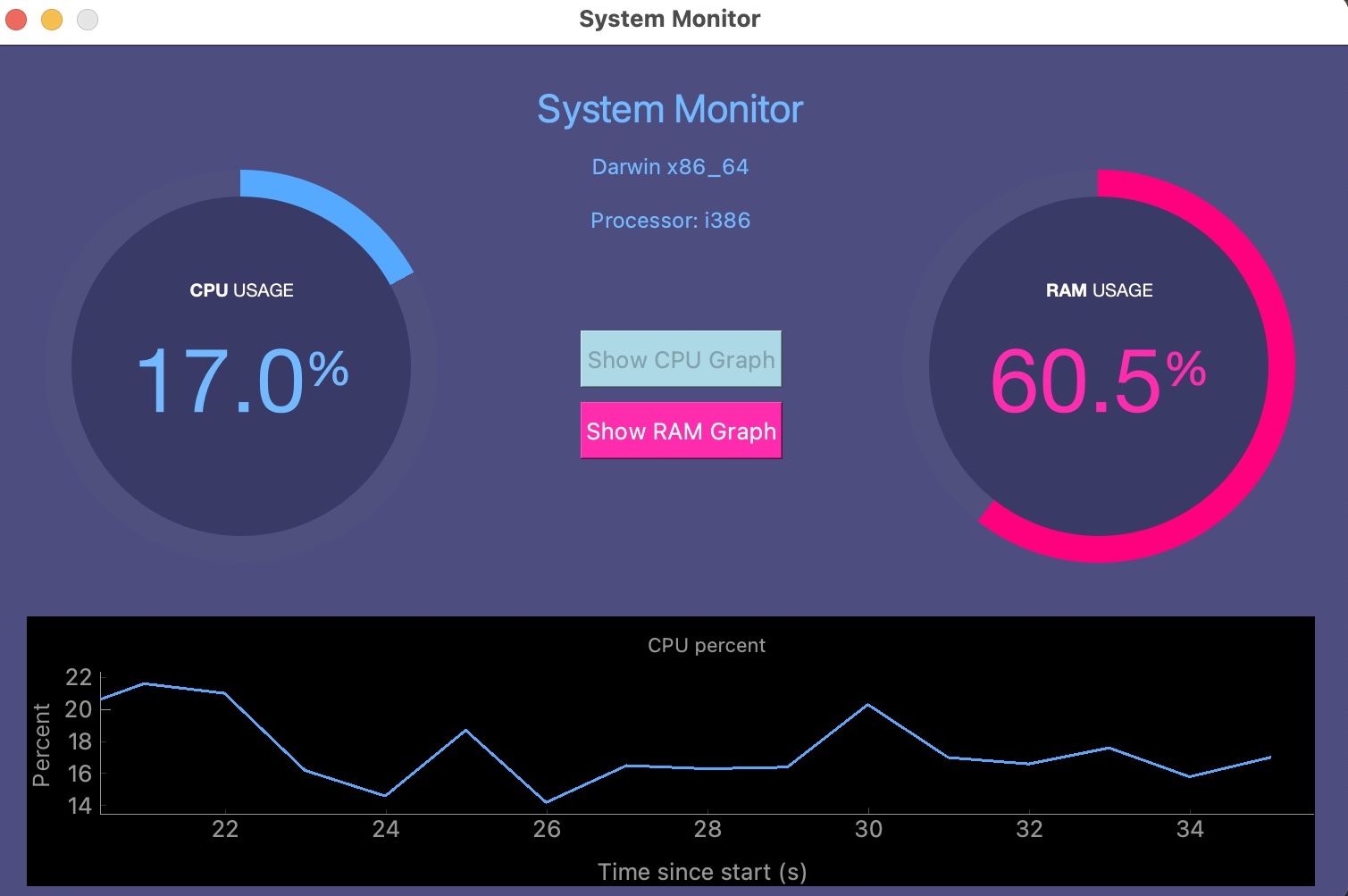## UTILITIES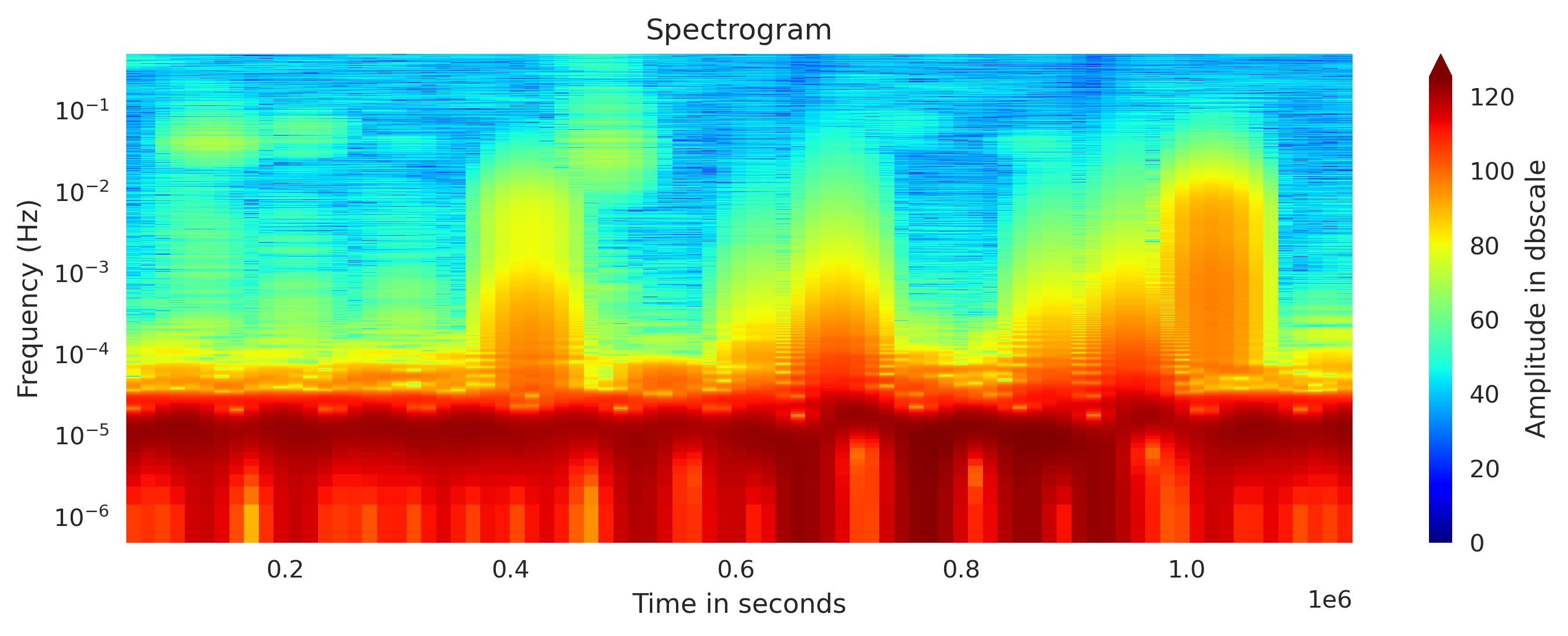## Concatenating daily seismic traces into one miniseed file

I concatenate the daily seismic traces for 15 days into one miniseed file for further analysis. Then I obtained the spectrogram of the 15 days seismic data. ...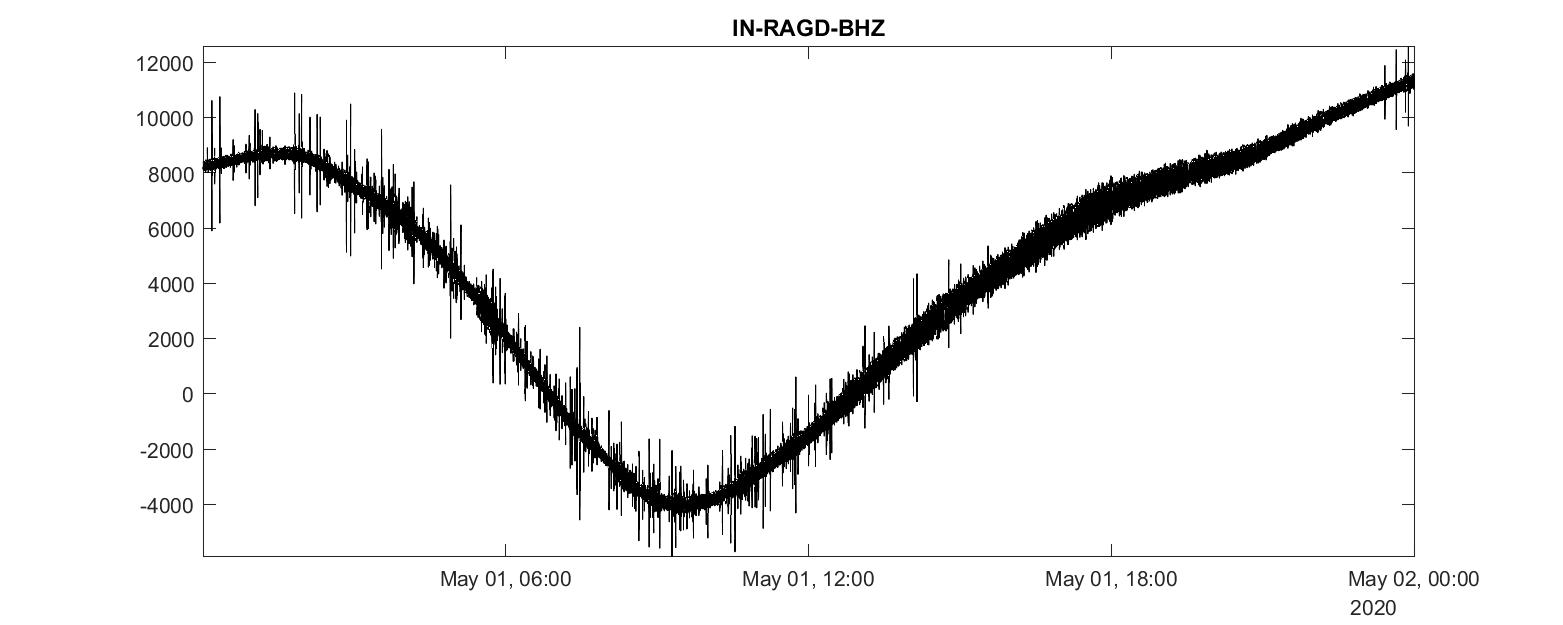## Analyzing miniseed seismic data in matlab

We will learn how to convert a mseed data file into mat format and then read and analyze it using MATLAB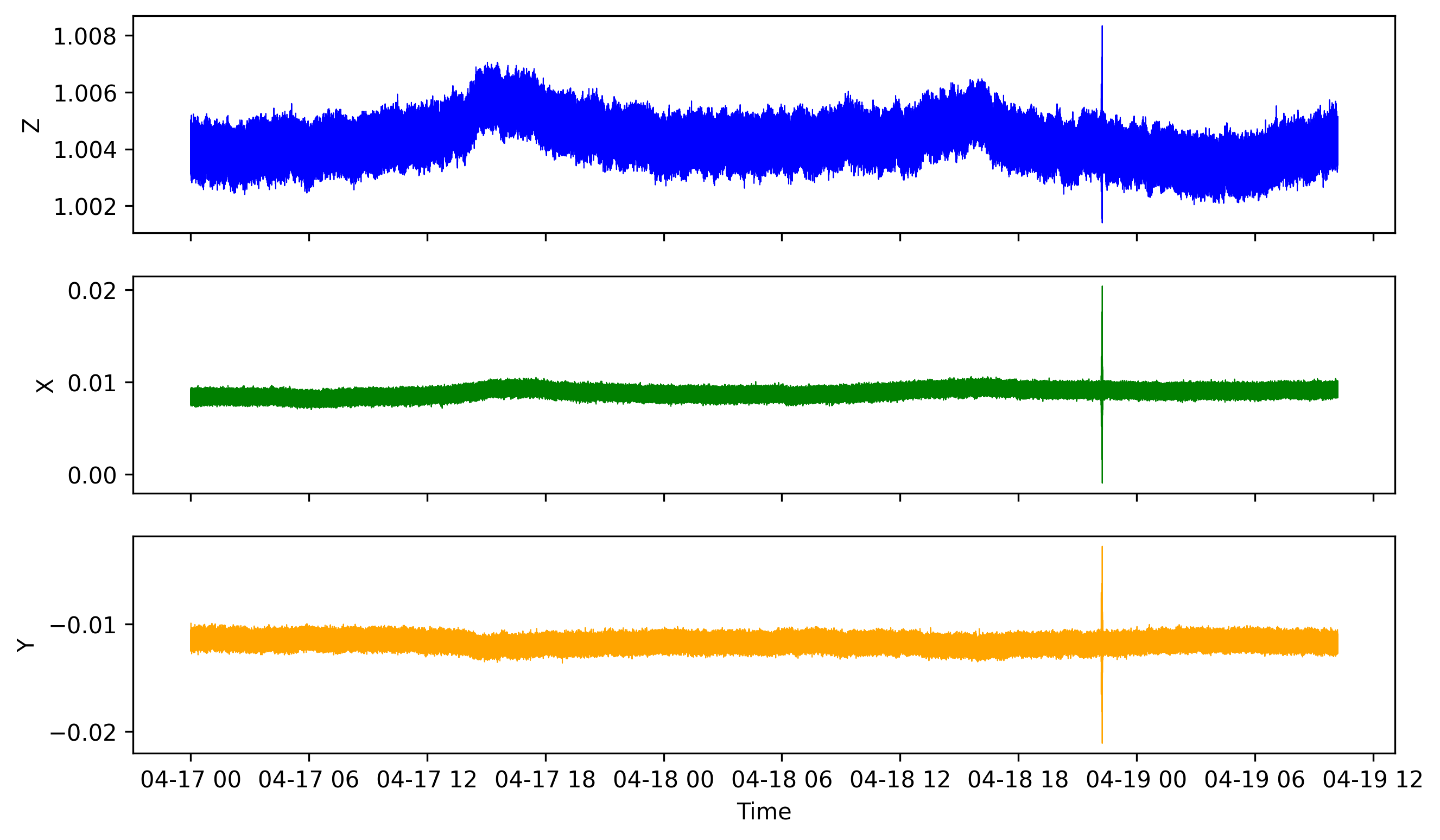## How to analyze a huge data file with pandas

We learn how to read huge csv file containing time series data by breaking it into chunks and then visualizing it with matplotlib## How to start using pandas immediately for earth data analysis

This tutorial gives a brief description of scientific computing using Pandas by introducing Series, DataFrame, Pandas common operations, methods, conditional...## Introduction to numpy for scientific computing

This tutorial gives a brief description of scientific computing using numpy by introducing arrays, methods, attributes, random numbers, indexing, broadcastin...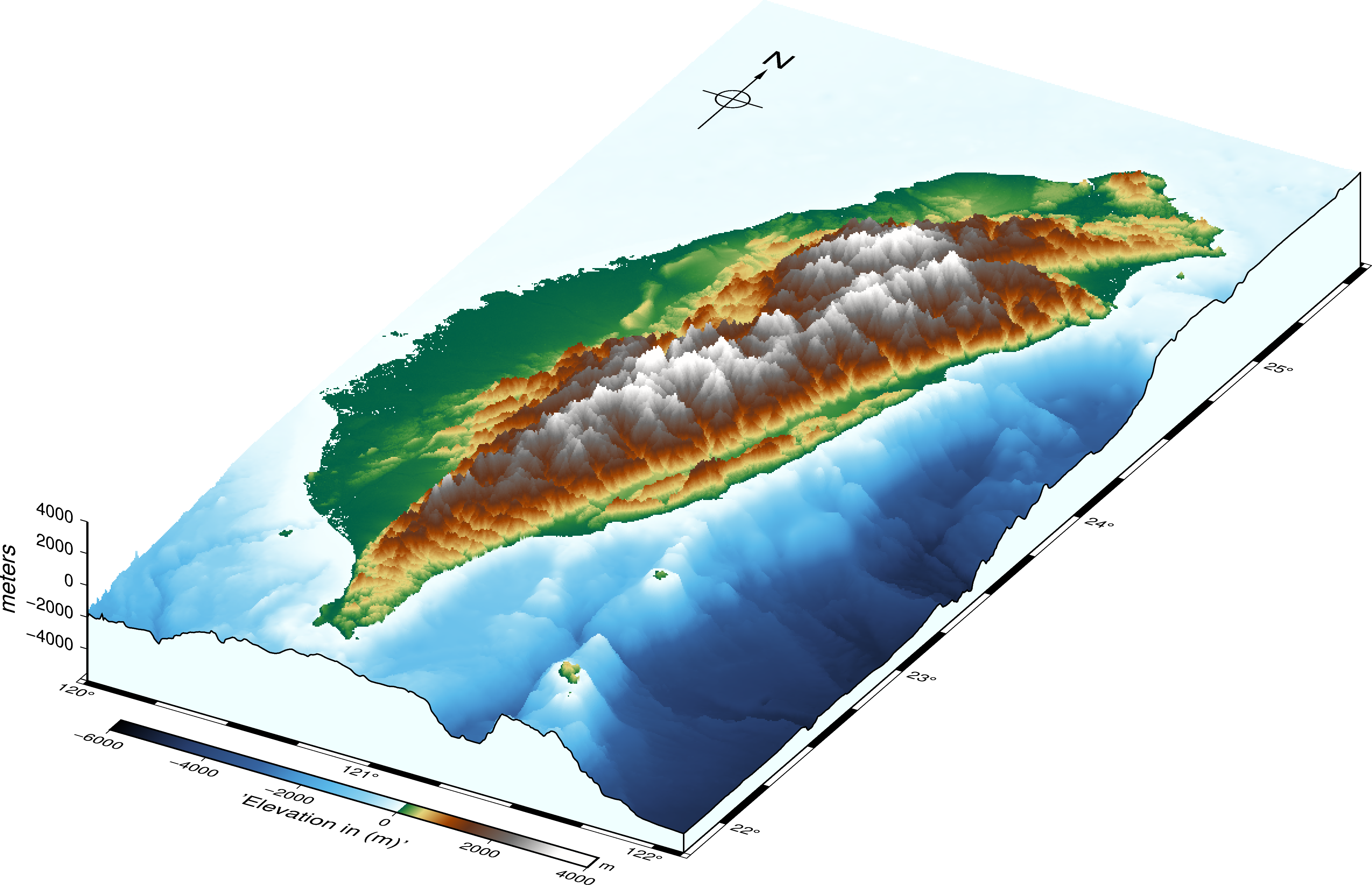## Three-dimensional perspective map of taiwan using gmt and pygmt

We learn how to make the three-dimensional map using both GMT and PyGMT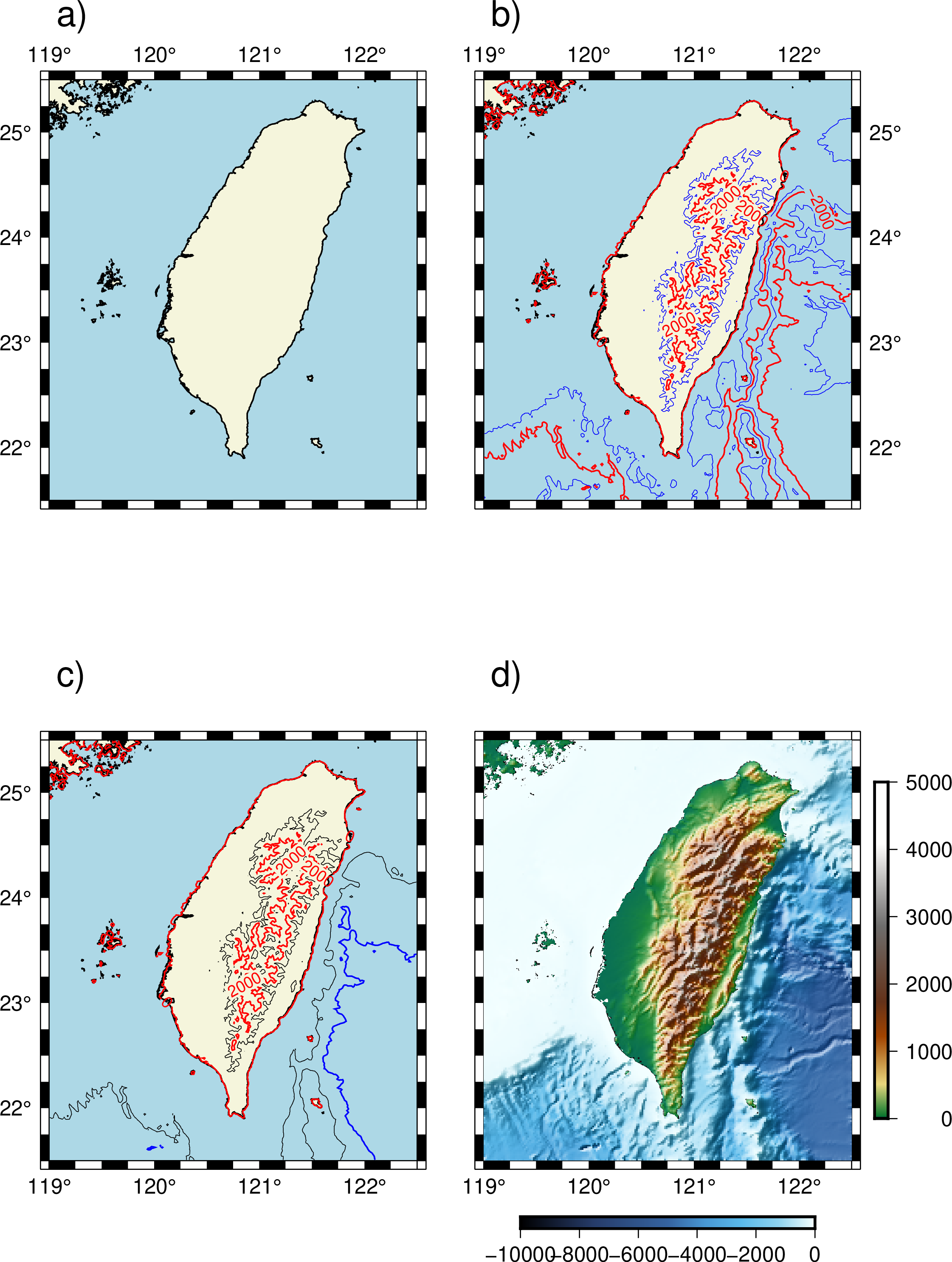## High-quality maps using the modern interface to the generic mapping tools

GMT or generic mapping tools have become synonymous with plotting maps in Earth, Ocean, and Planetary sciences. It can be used for processing data, generatin...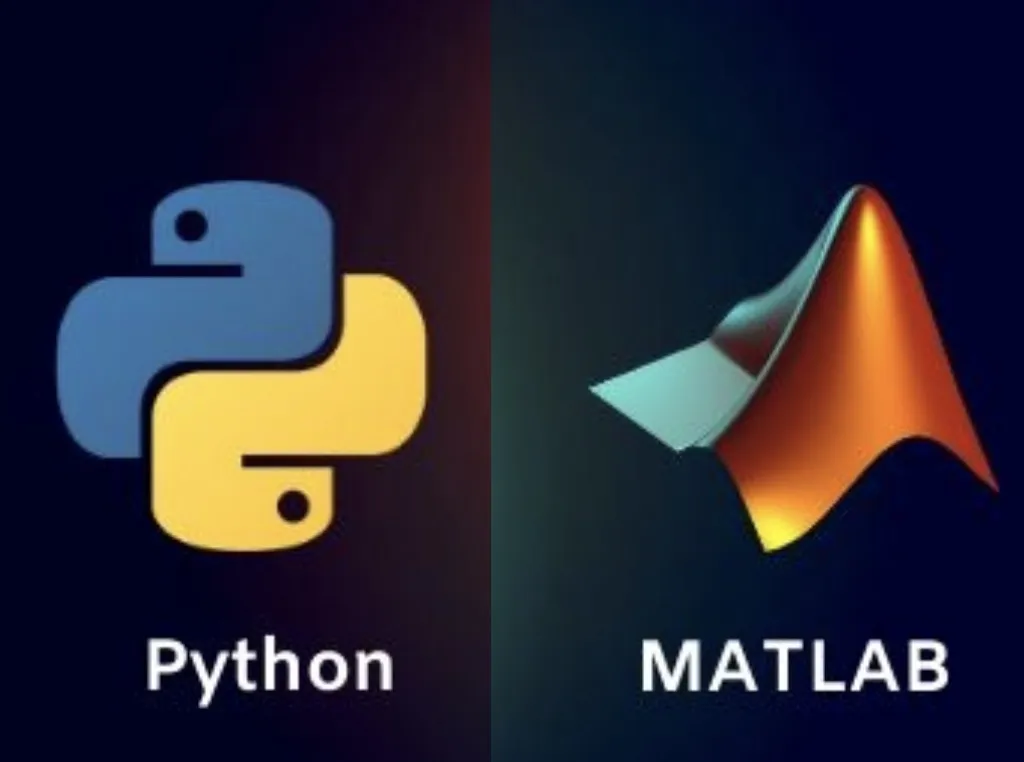## Easily integrate custom functions in matlab with python

How can we use the MATLAB functions in Python? MATLAB implementation are usually reliable as it is developed by the professionals. But the advantages of usin...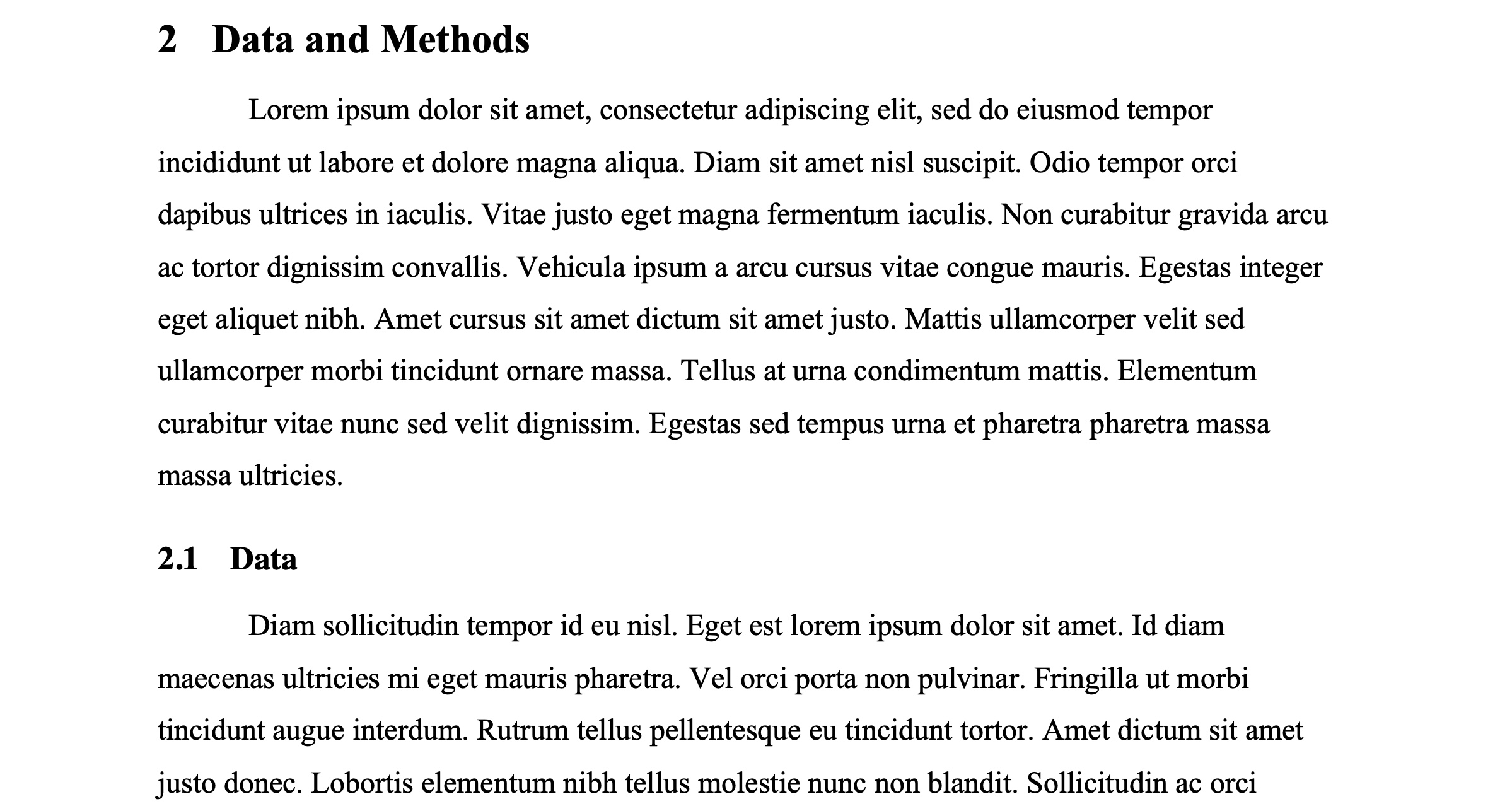## Writing and formatting a scientific manuscript in microsoft word

less than 1 minute read      UTILITIES

If you are ready to use the Microsoft Word as your favourite tool for writing your awesome scientific thoughts and ideas into a manuscript, then I would like...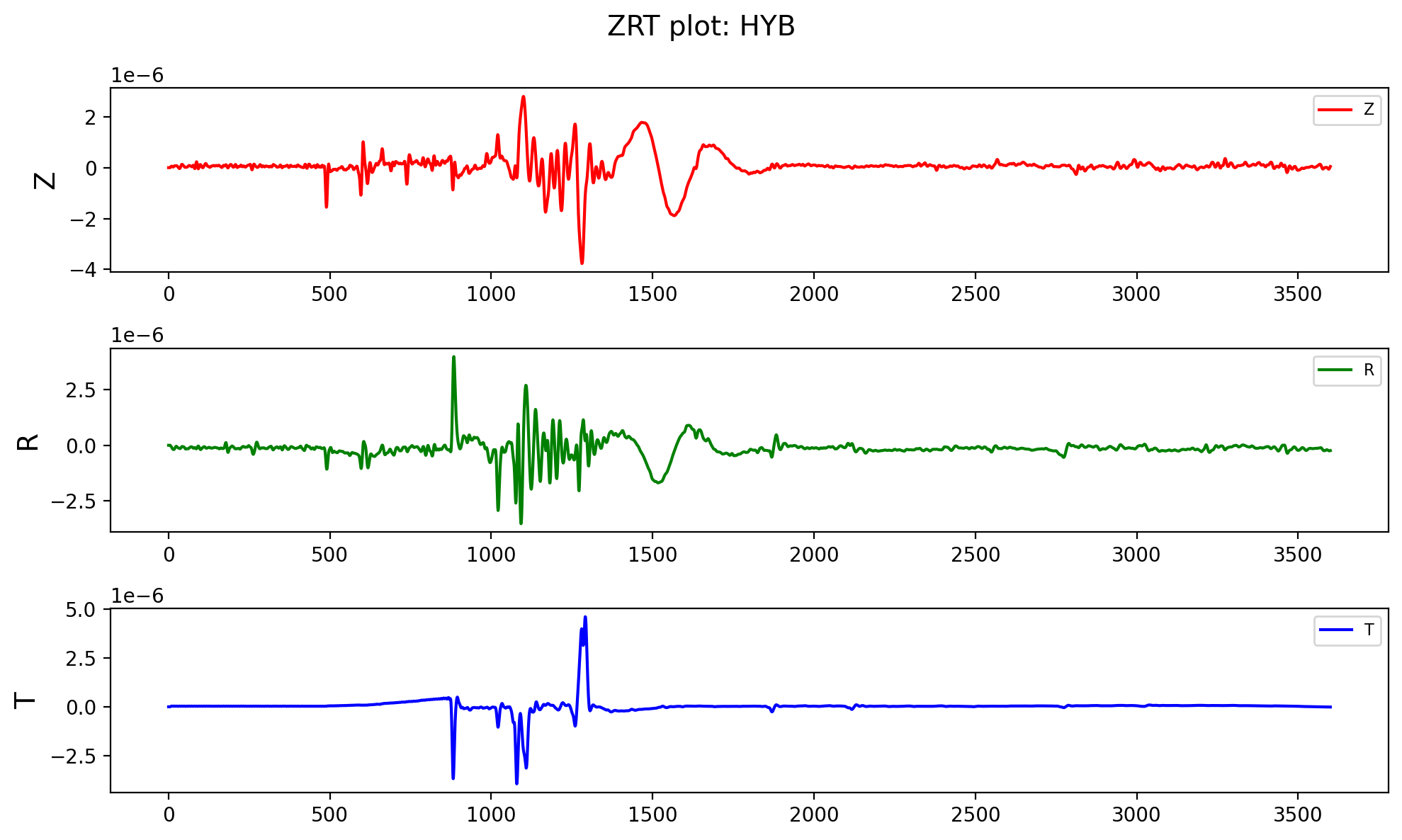## Write ascii data to mseed file using obspy

In this post, I will read a ASCII file whose first few lines contains the header information and then the three-component data. I will read using the pandas ...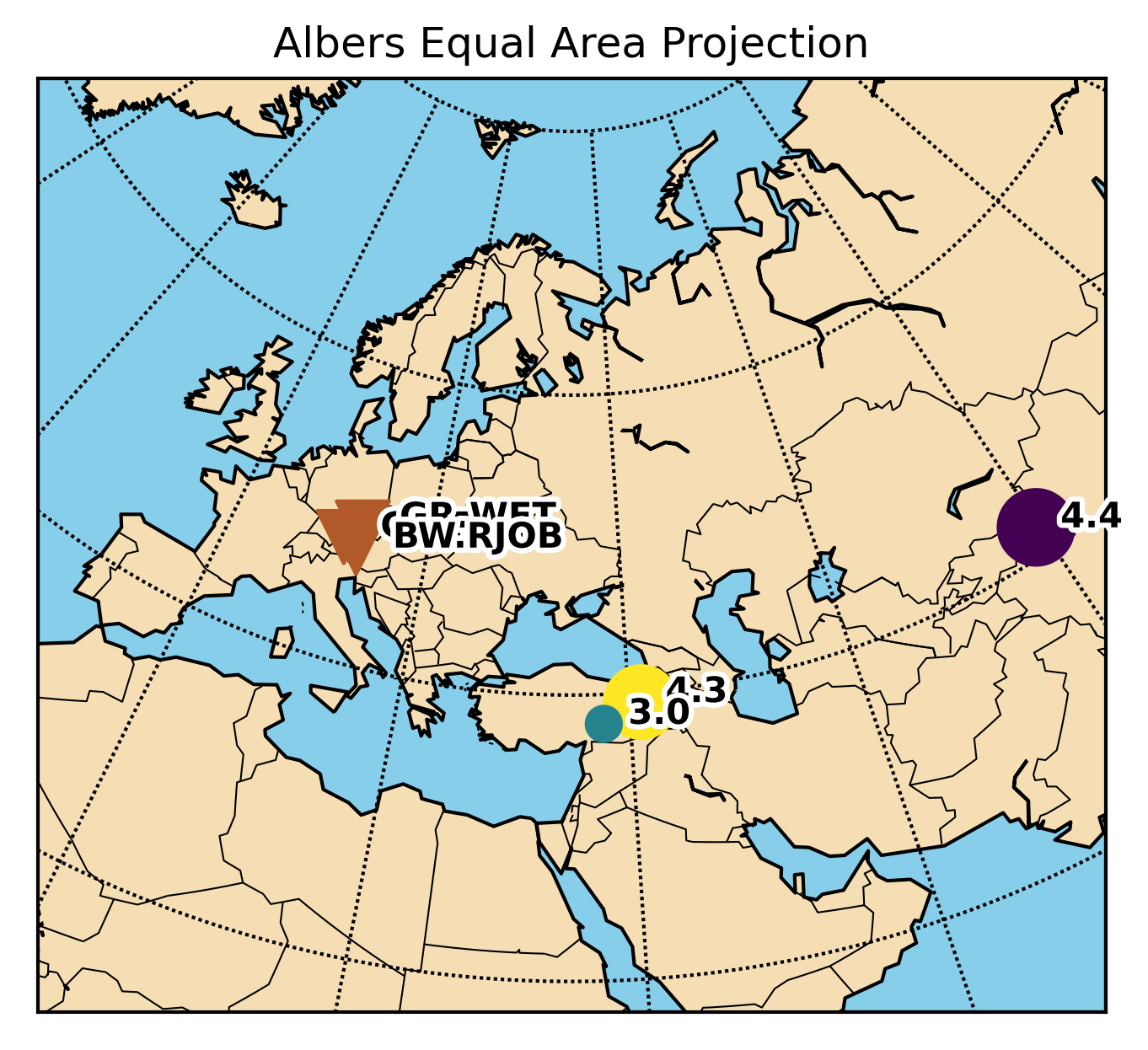## Working with obspy and basemap

This post is aimed to resolve the issues regarding the conflicts of using obspy and basemap libraries together.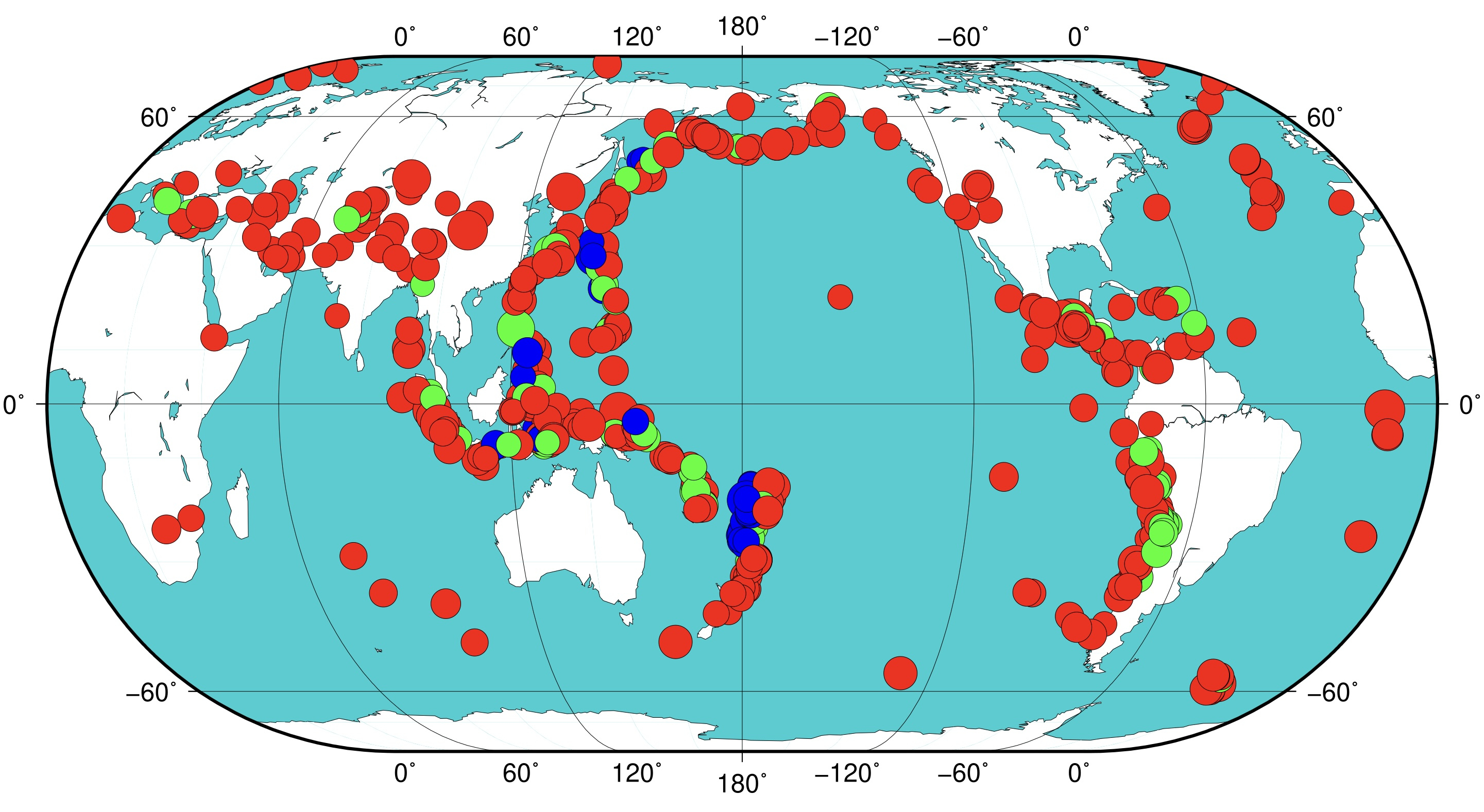## Gmt tutorial for beginners

The Generic Mapping Tools is widely used across Earth and Planetary and other fields of studies to process data and generate high-quality illustrations. This...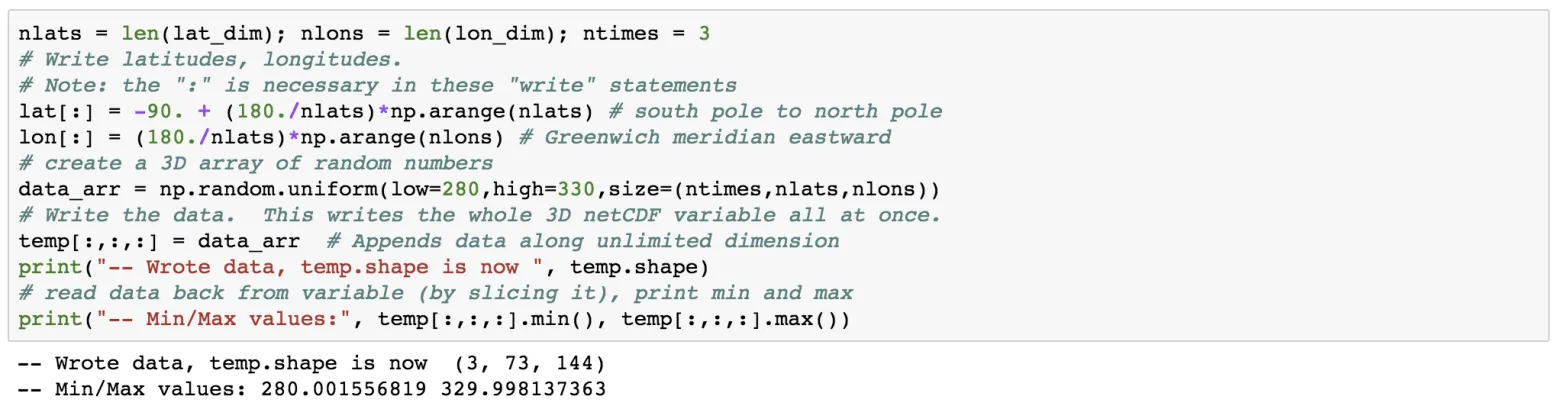## Writing netcdf4 data using python

NetCDF file format has been designed for storing multidimensional scientific data such as temperature, rainfall, humidity, etc. In this post, we will see how...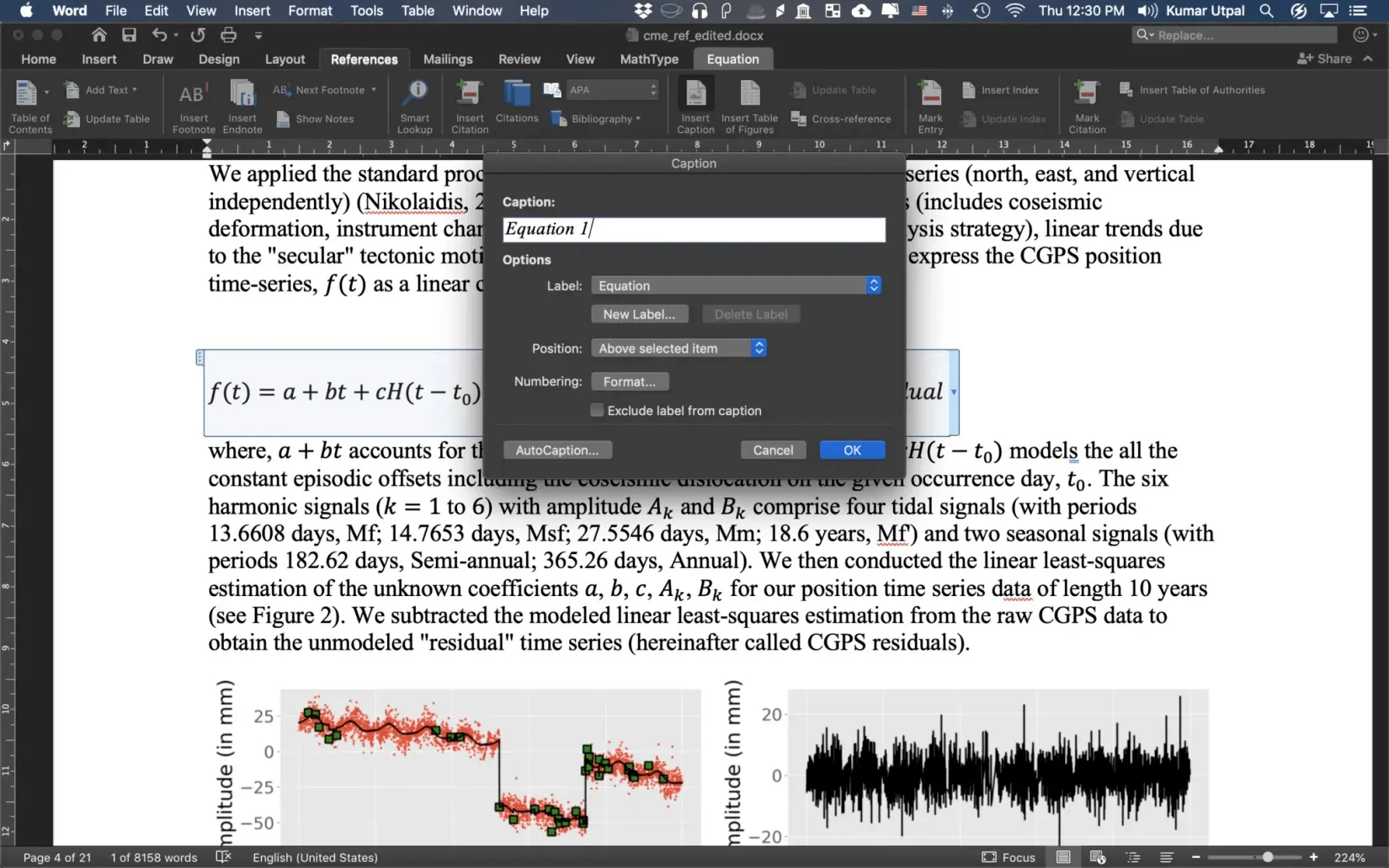## How to insert equation numbering in ms word

It is essential to insert equation numbers in your thesis and/or any scientific paper. In this post, I will show you some of the easiest ways to insert equat...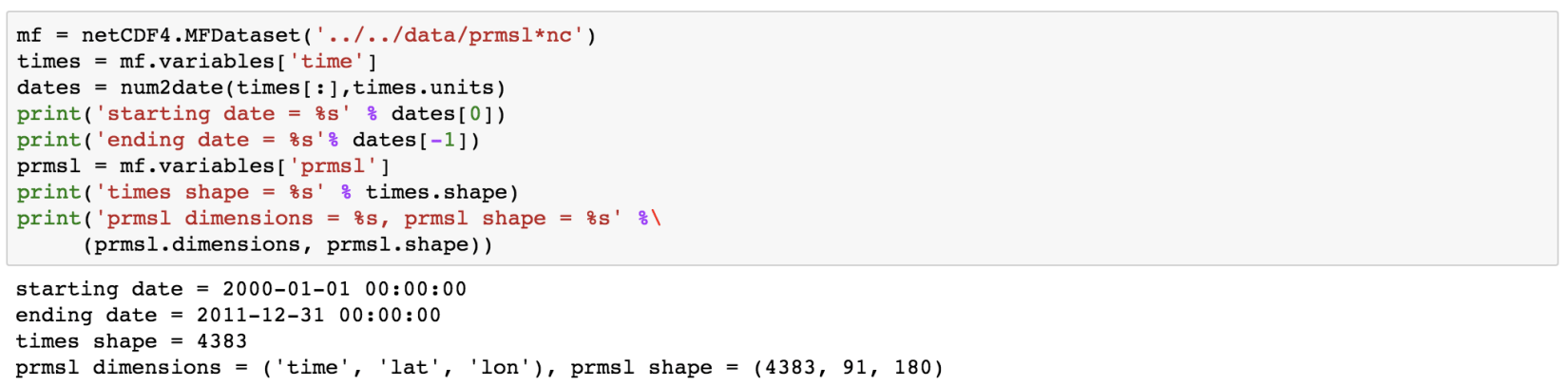## Reading netcdf4 data in python

In Earth Sciences, we often deal with multidimensional data structures such as climate data, GPS data. It ‘s hard to save such data in text files as it would...## Pygmt: high-resolution topographic map in python

A simple tutorial on how to plot high resolution topographic map using GMT tools in Python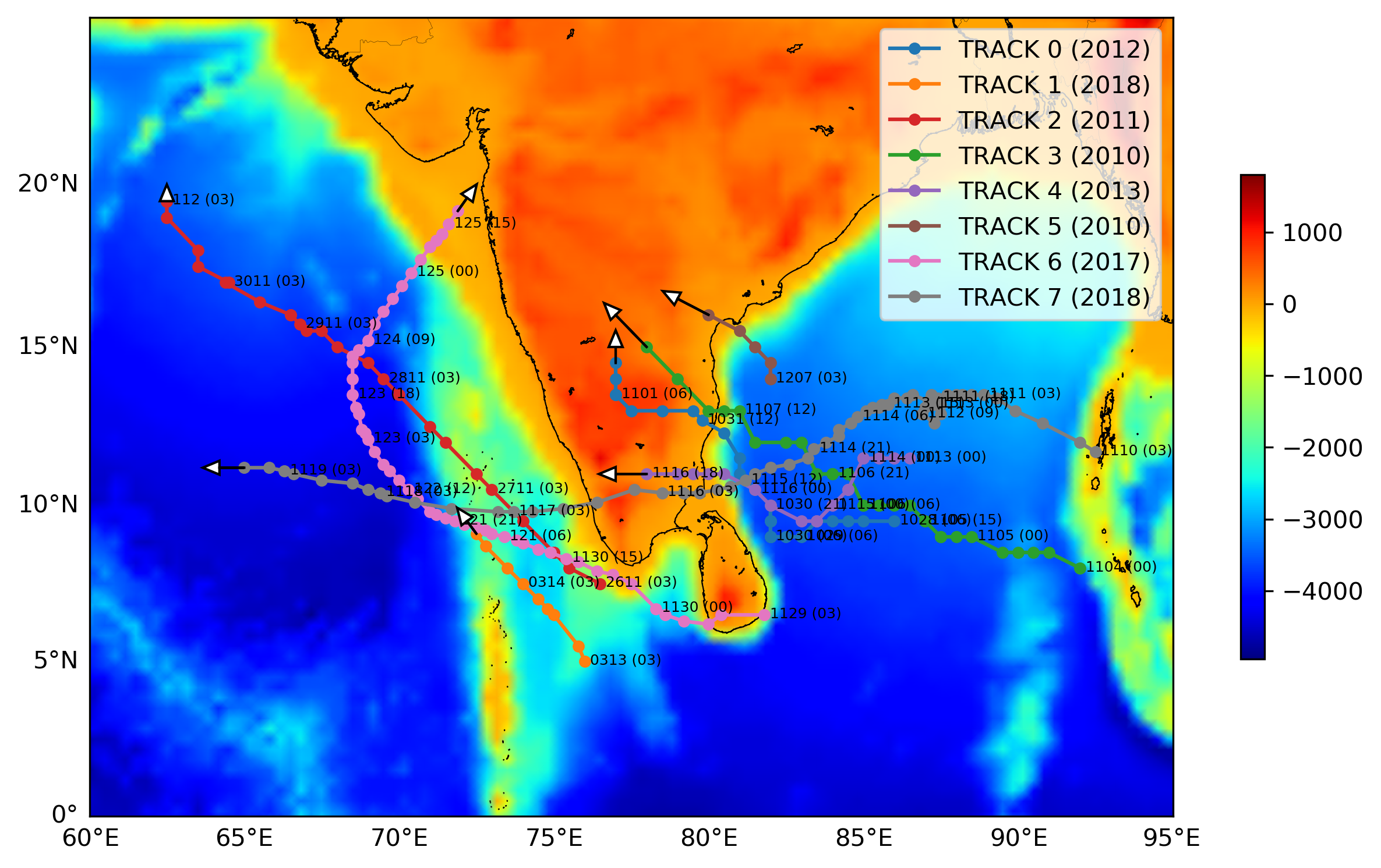## Plotting track and trajectory of tropical cyclones on a topographic map in python

Short demonstration of how to plot the track or trajectory of a hurricane on a map. Codes are included.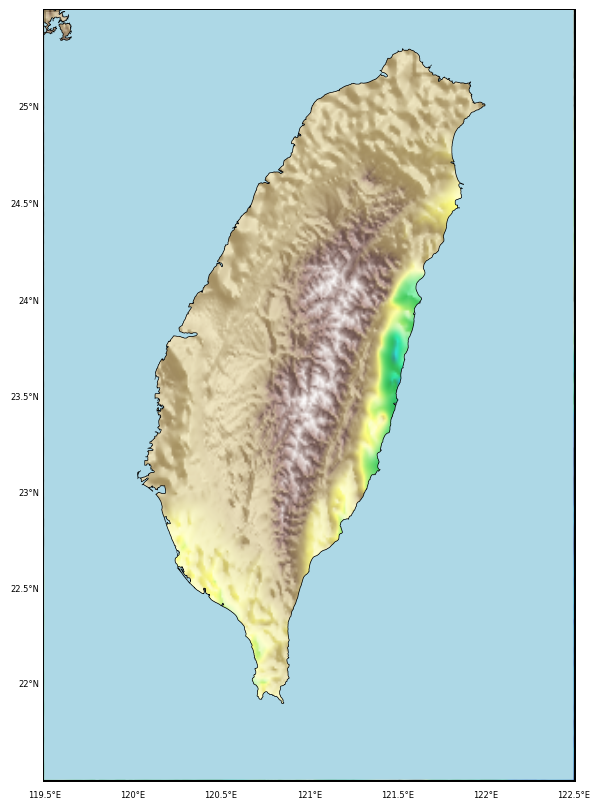## Topographic map clipped by coastlines in python

This post demonstrate how to use Python to set up clip topographic map based on coastlines.## Topographic map with shading in python

Generating GMT style shaded relief map in Python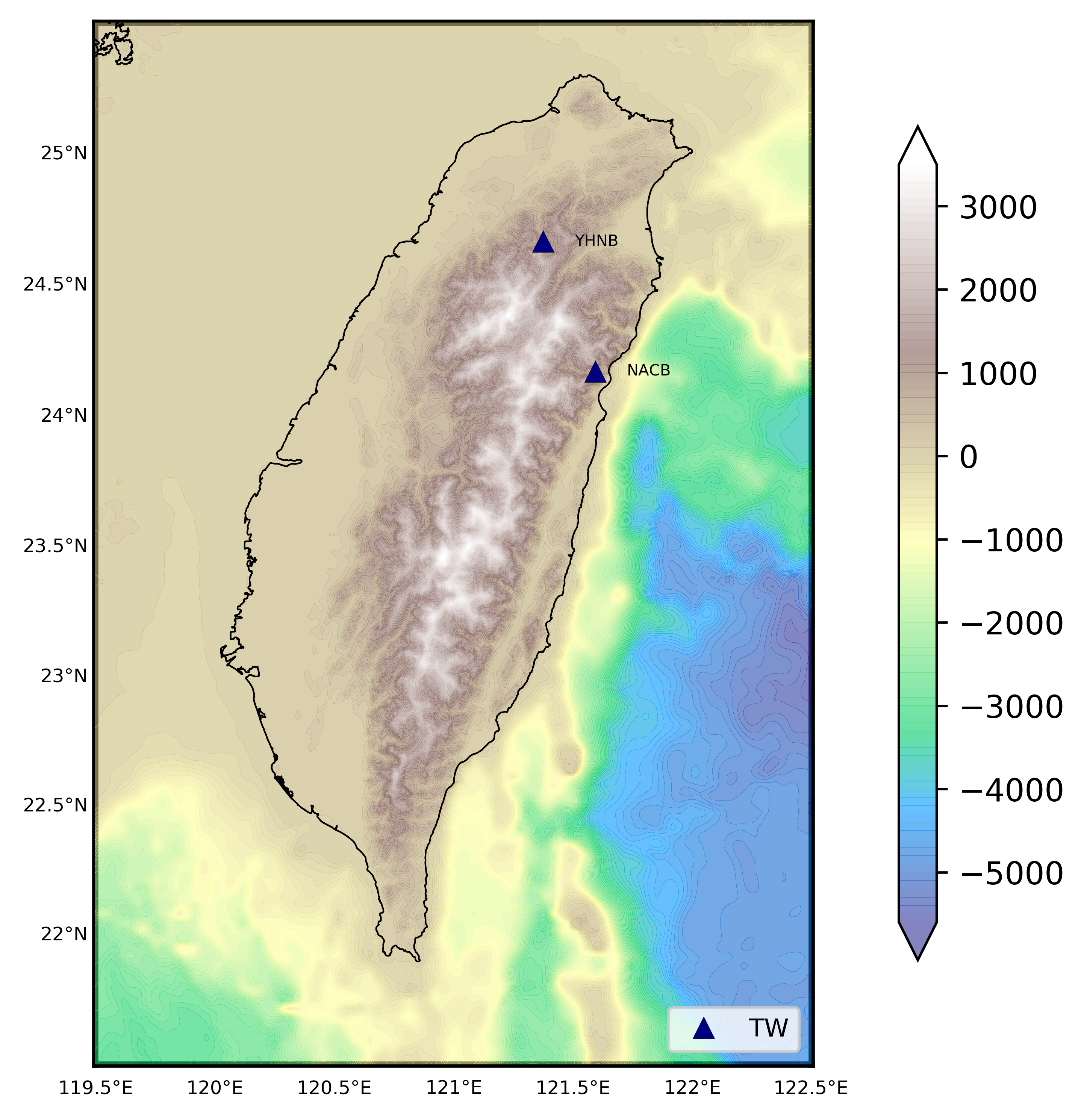## How to plot topographic station map in python

We read the station information from a file to plot the 1 arc-minute relief map## Plotting 1 arc-minute global relief map in python

Plotting 1 arc-minute topographic map in python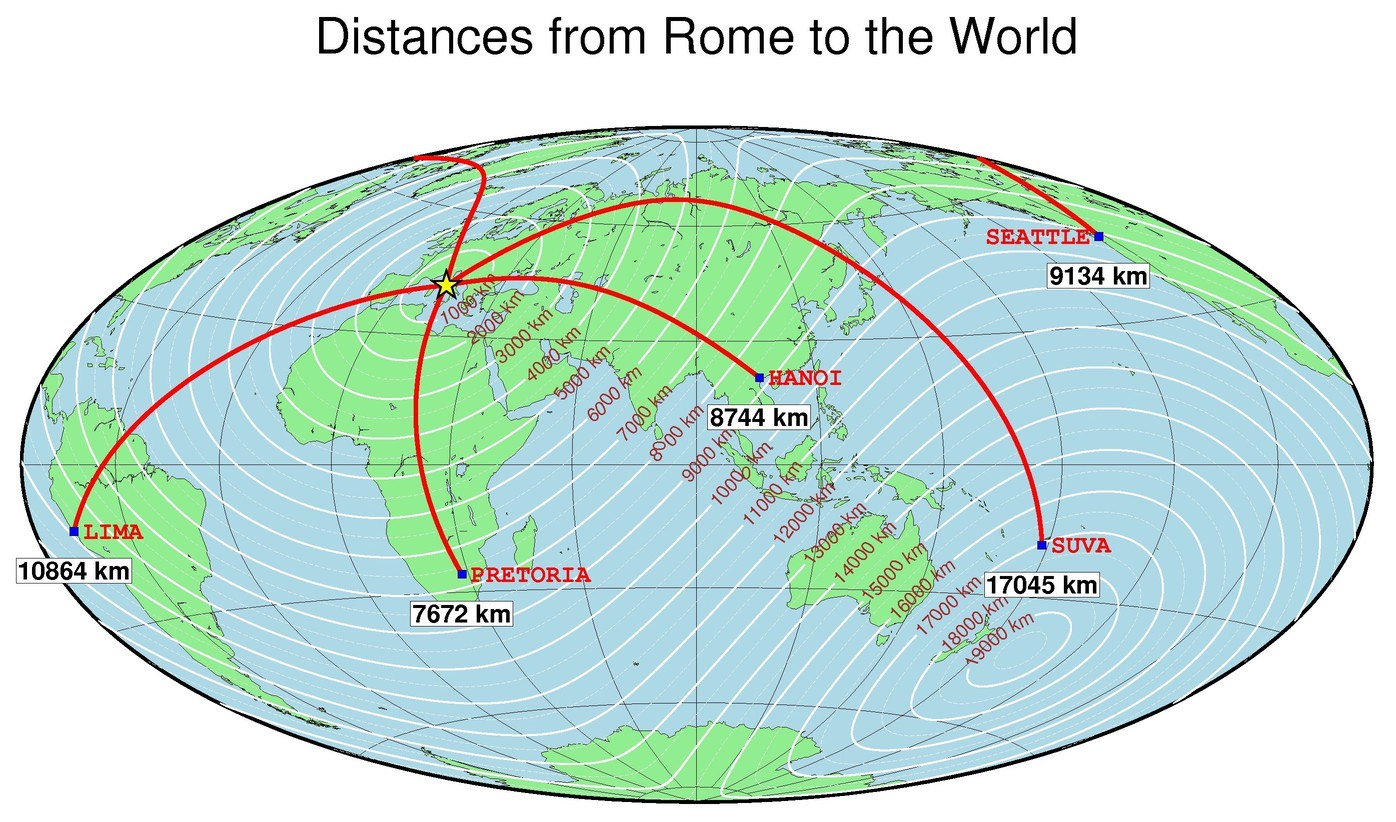Plotting publishable quality maps using generic mapping tools (GMT)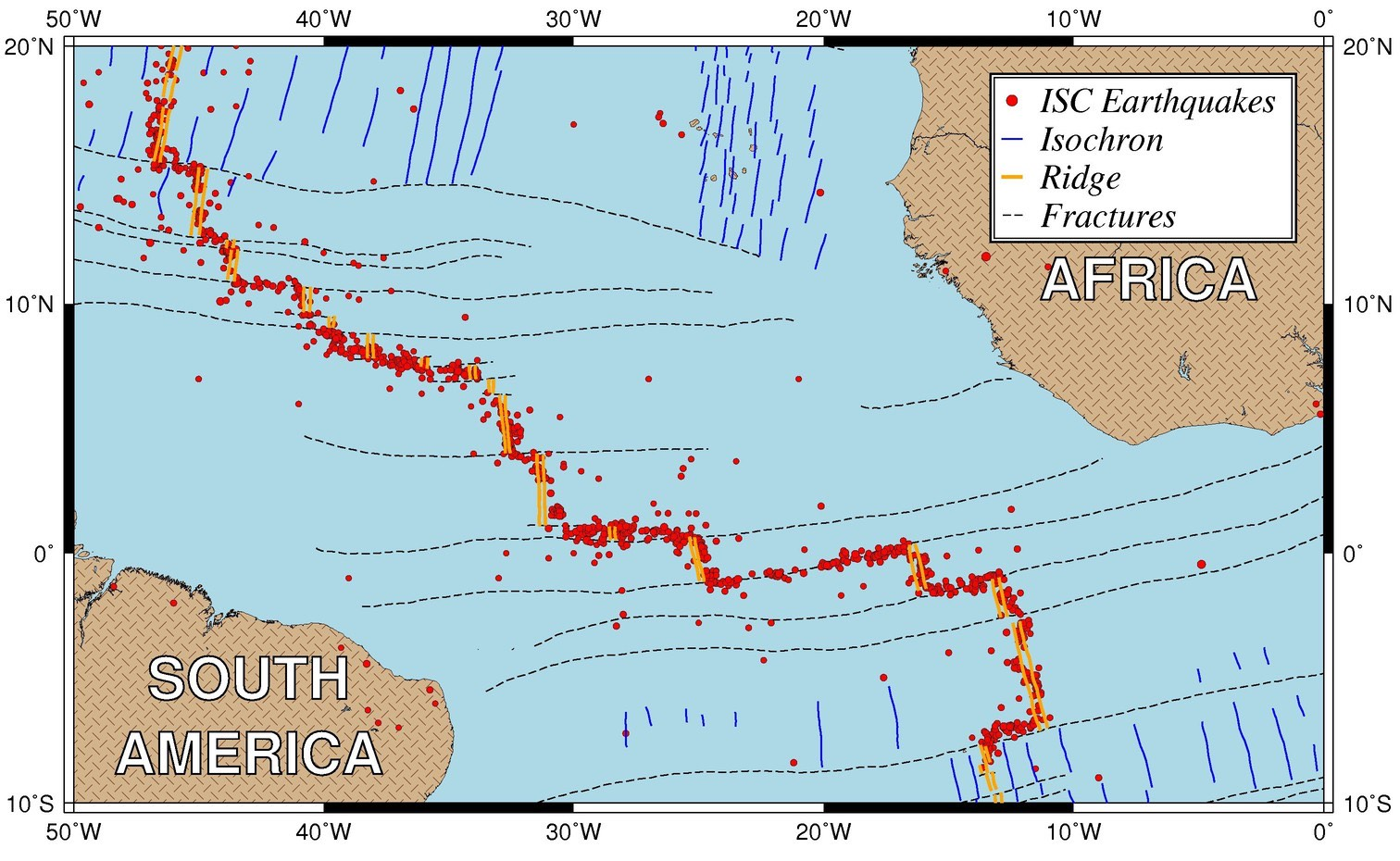Plotting publishable quality maps using generic mapping tools (GMT)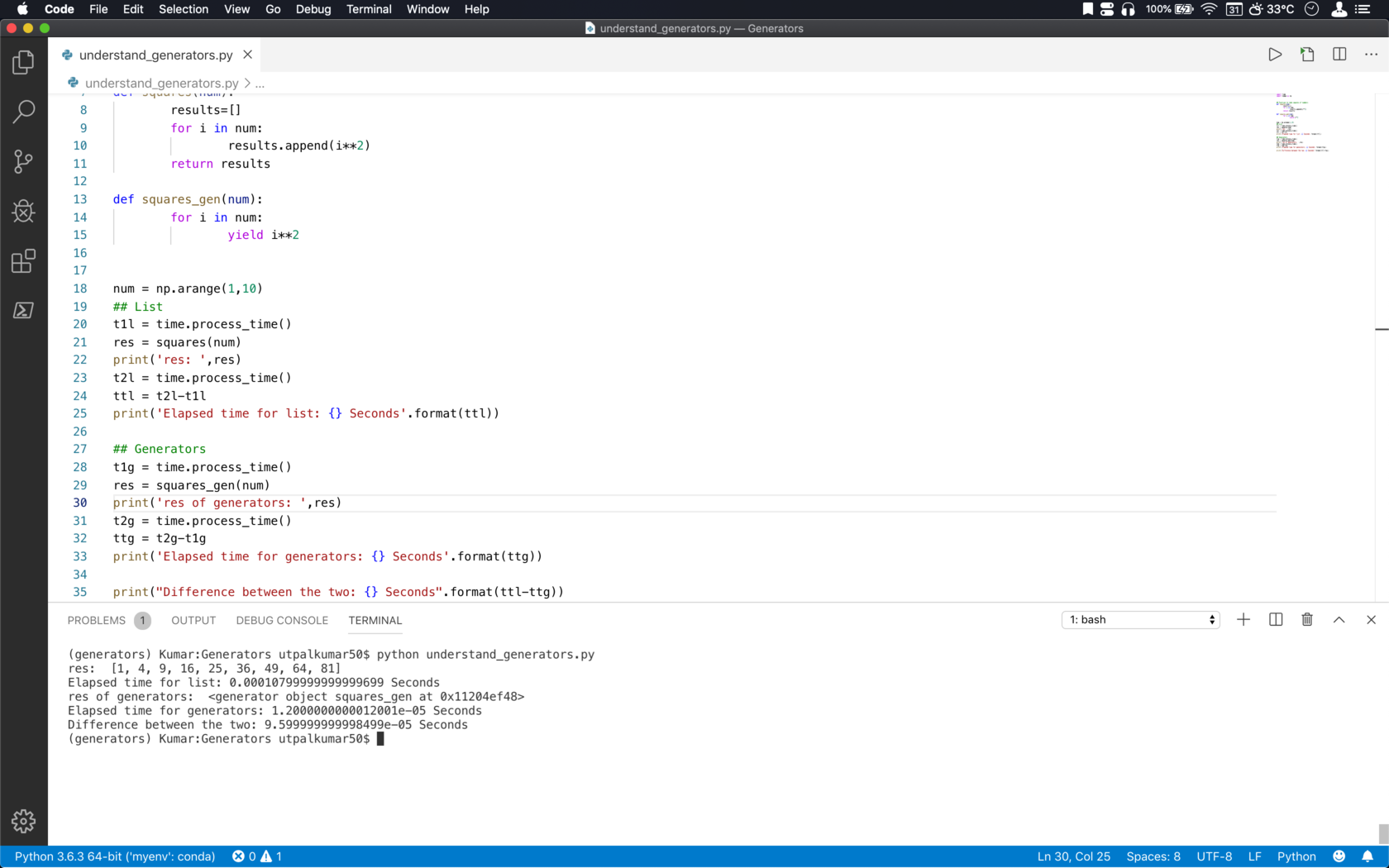## Why we should use generators? [python]

Generators don’t hold the entire result in memory. It yields one result at a time.

## GEOPHYSICS## Wavelet analysis applied to the real dataset in a quick and easy way

An introduction to the wavelet analysis for a real geophysical data set. I compared the analysis to the Fourier analysis. Codes included!## Genetic algorithm: a highly robust inversion scheme for geophysical applications

An introduction to the basics of genetic algorithm along with a simple numerical example and solution of an earthquake location problem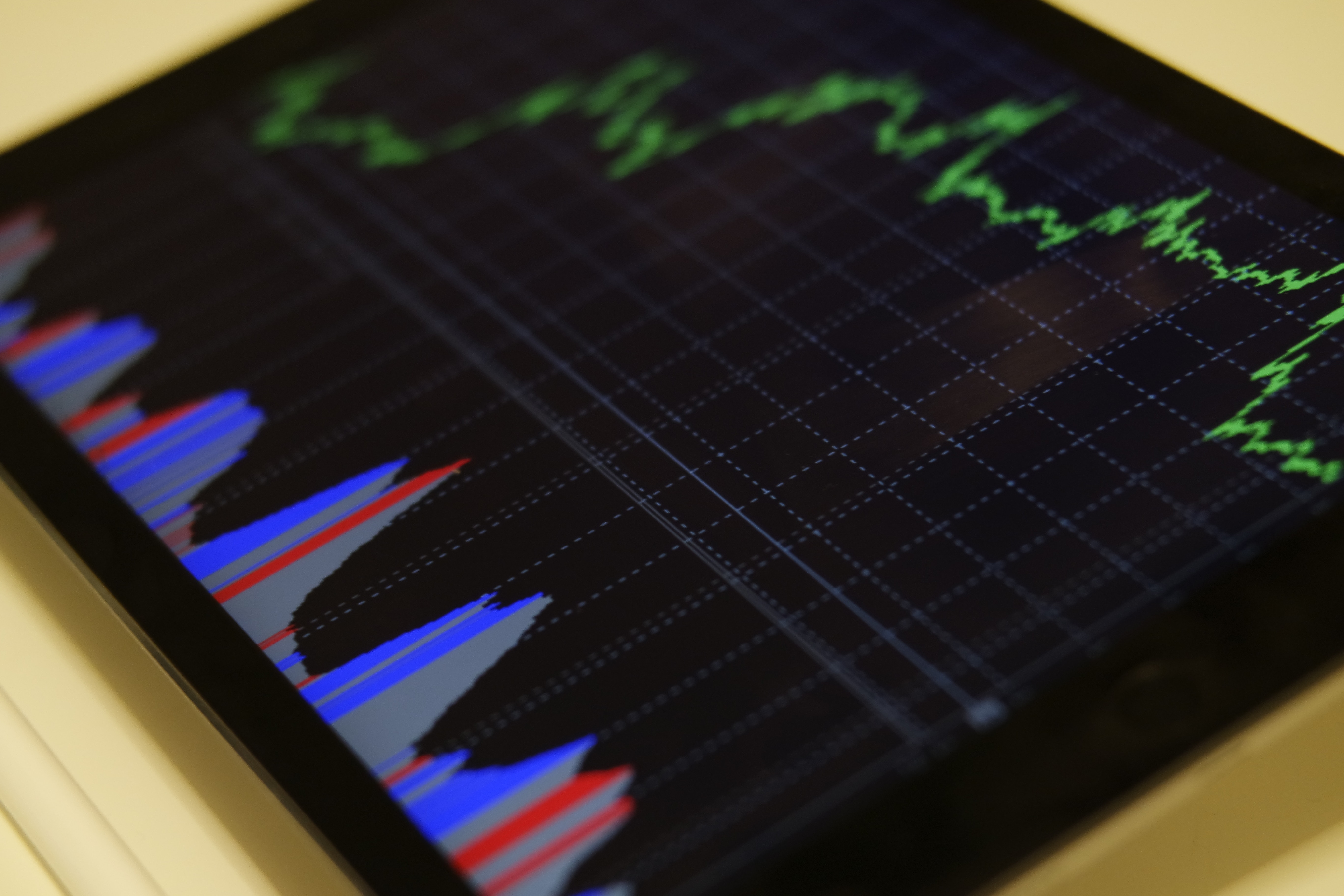## The easy way to compute and visualize the time & frequency domain correlation

In geophysics, it is important to understand and identify the complex and unknown relationships between two time-series. Cross-correlation is an established ...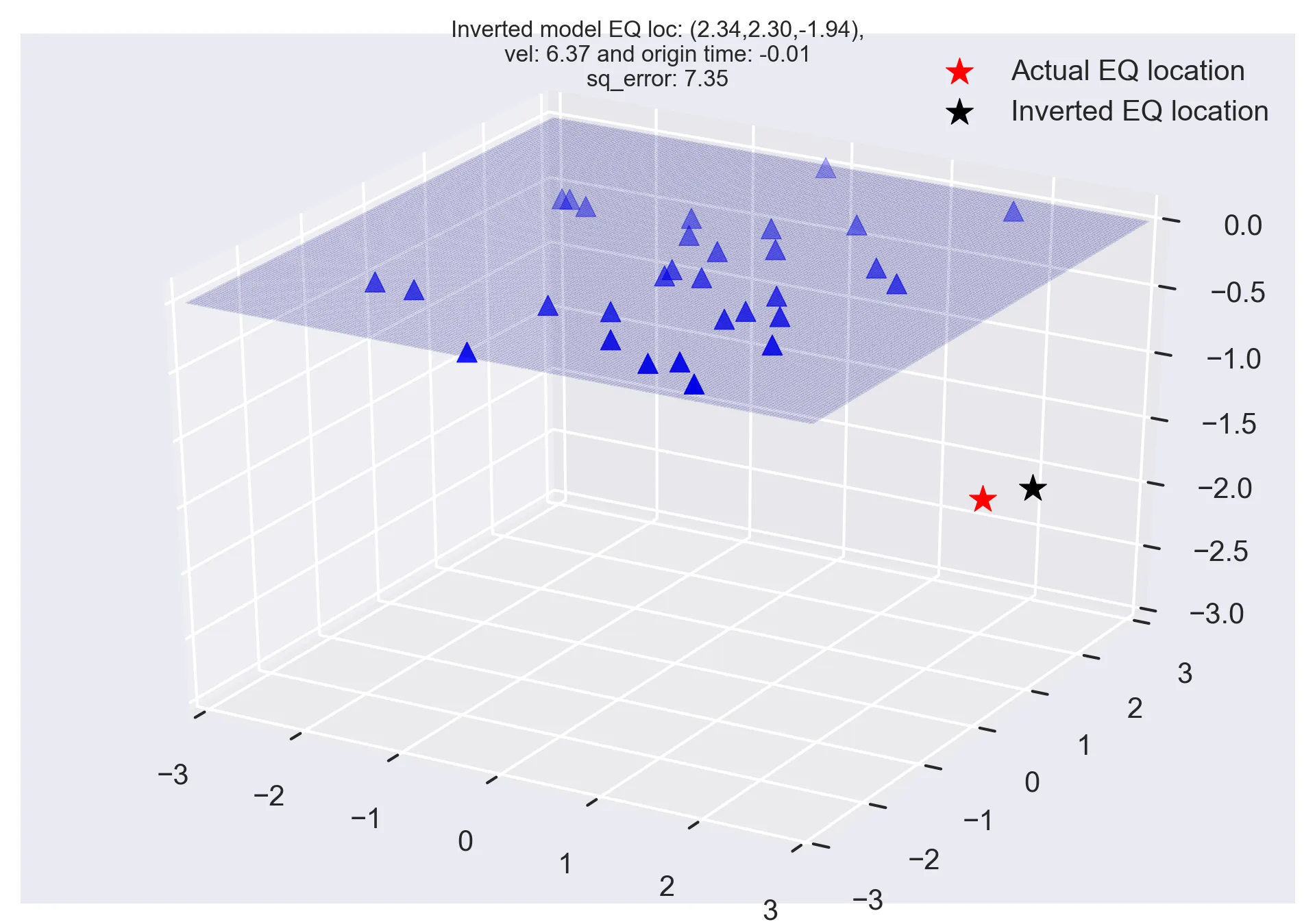## Monte carlo methods and earthquake location problem

The common geophysical problems most often have multimodal objective function with many possible minima. In this post, we will look into the Monte Carlo meth...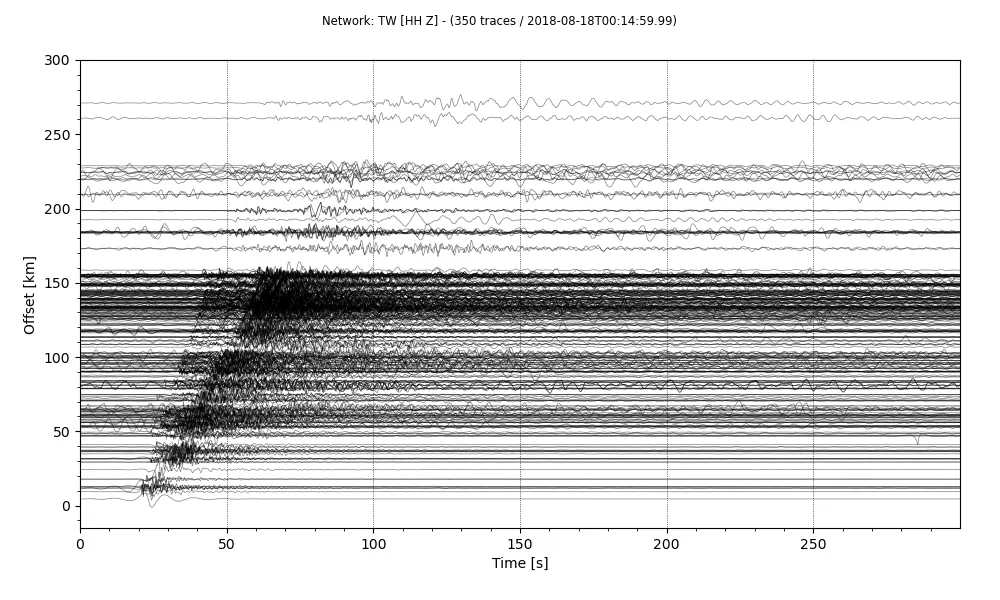## Plotting a record section using obspy

Quickly plot record section of a stream using Obspy. I will introduce you how to make a stream from a set of SAC data, plot the record section and store it a...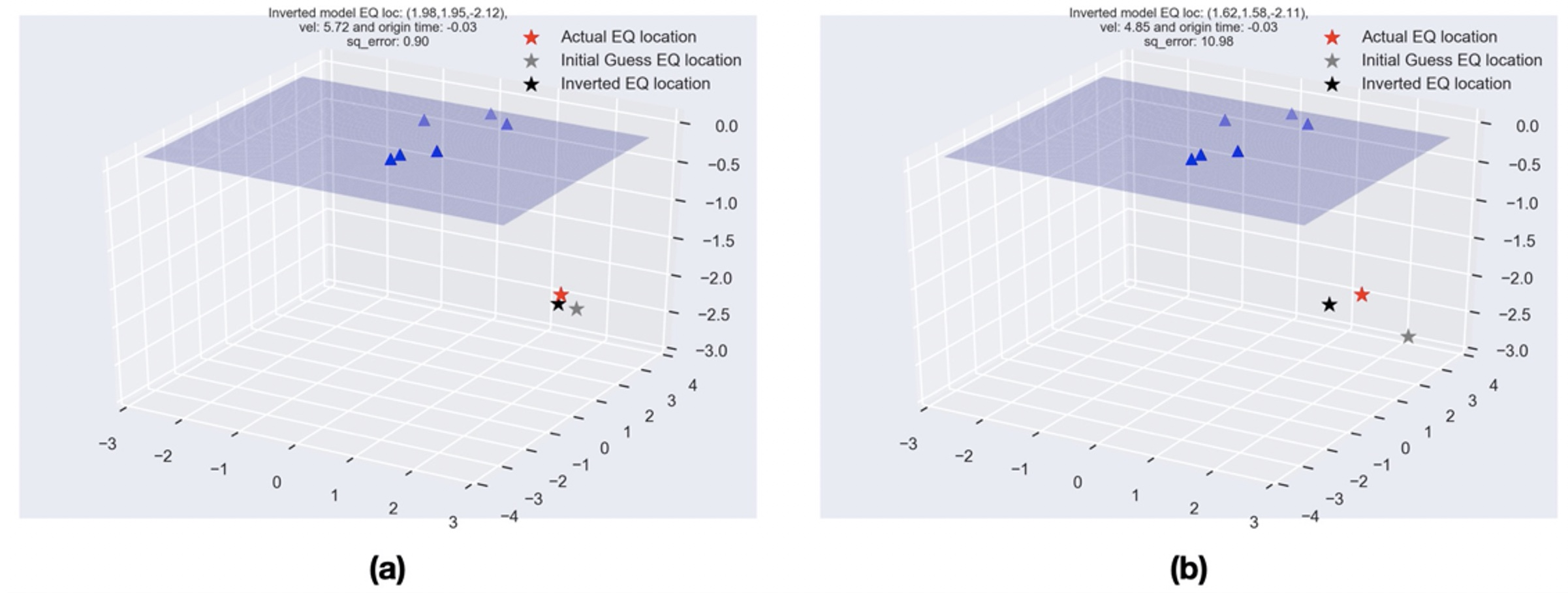## Least-squares method in geosciences

Least-squares method is a popular approach in geophysical inversion to estimate the parameters of a postulated Earth model from given observations. This meth...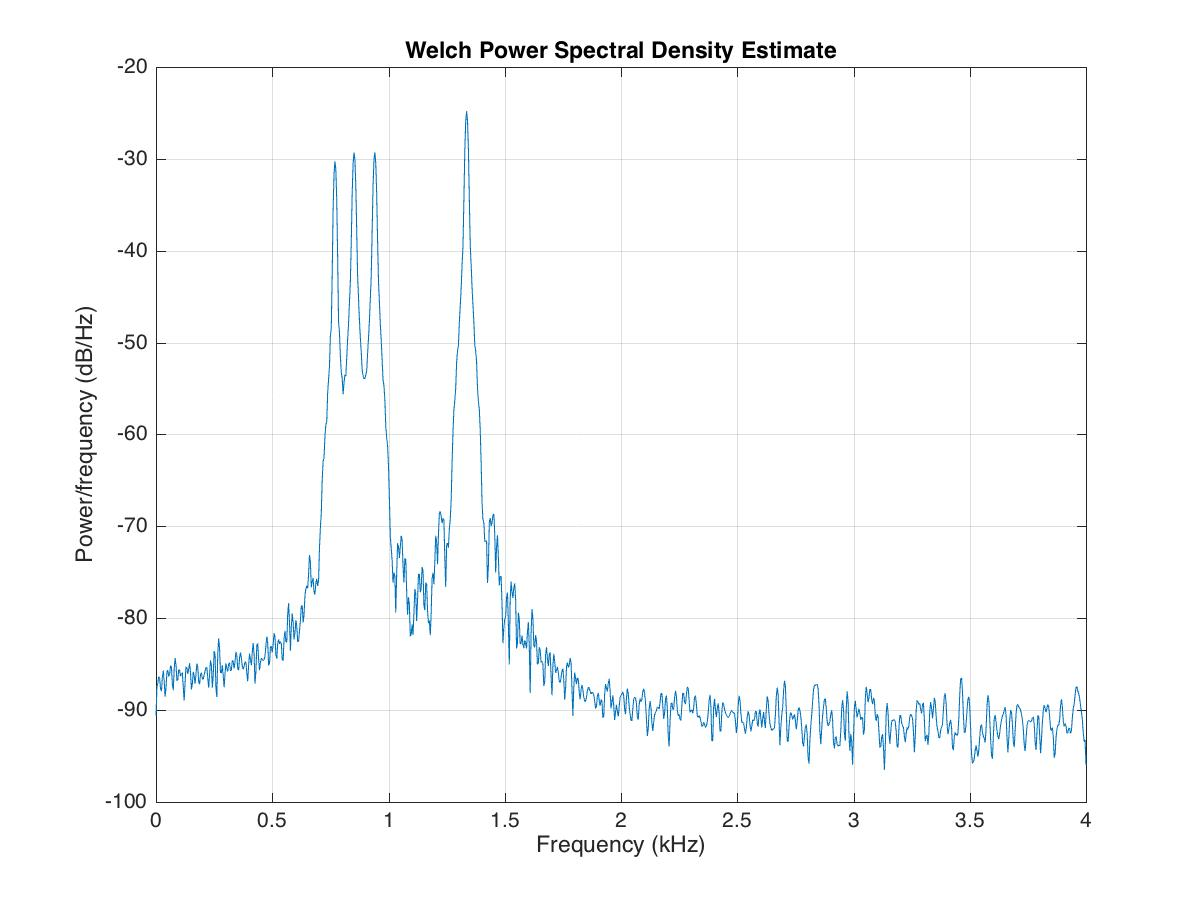## Time-frequency analysis in matlab

A signal has one or more frequency components in it and can be viewed from two different standpoints: time-domain and frequency domain. In general, signals a...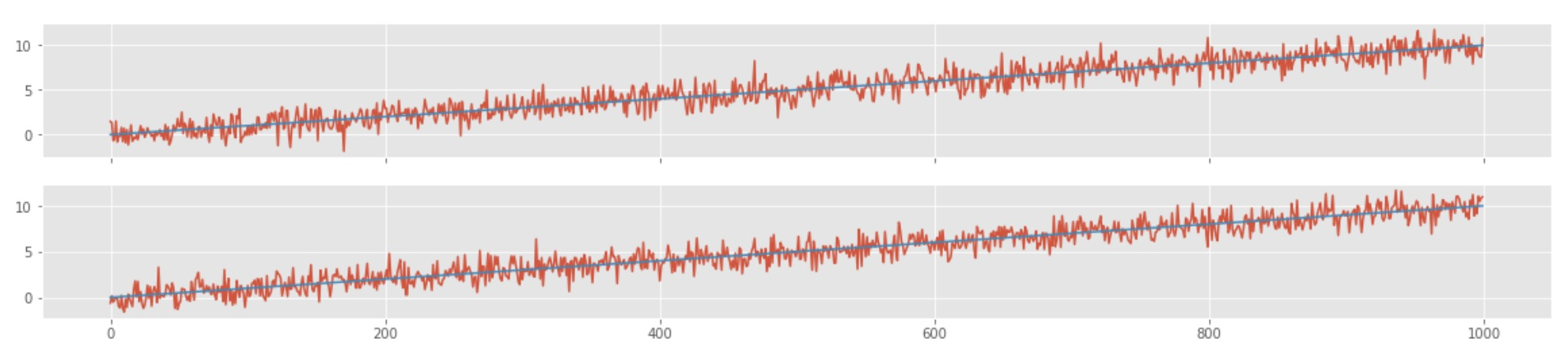## How to avoid common mistakes in analyzing correlations of two time-series

Most often data analyst consider correlation between two time-series as a causation effect. Two time-series are correlated that does not imply that one cause...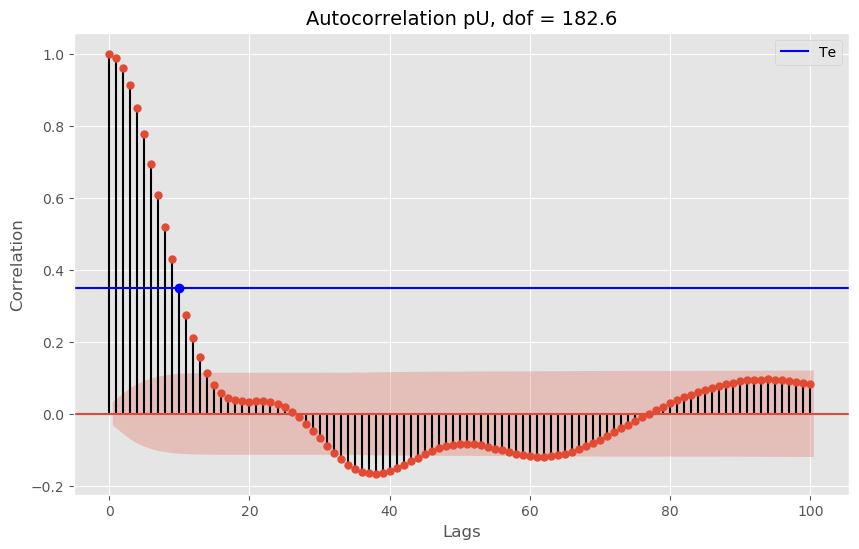## Estimation of the degrees of freedom for time series

Numerical tests to understand the degrees of freedom in time series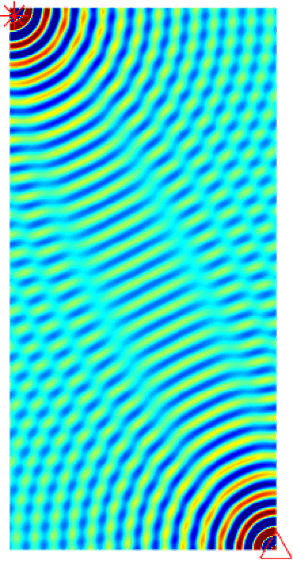## Numerical tests for seismic resolution

Seismic resolution and fidelity are the two important measures of the quality of the seismic record and the seismic images. Seismic resolution quantifies the...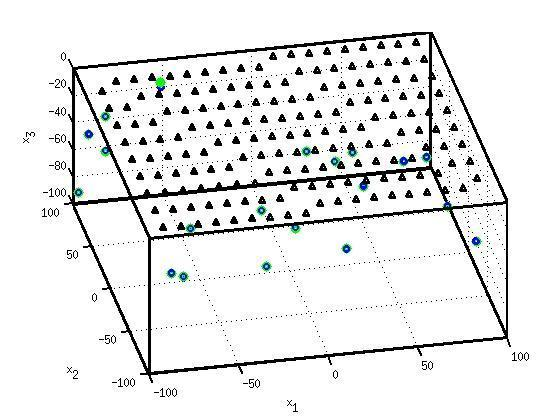## Locating earthquakes using geiger’s method

Simple earthquake location problem and its solution using Geiger’s method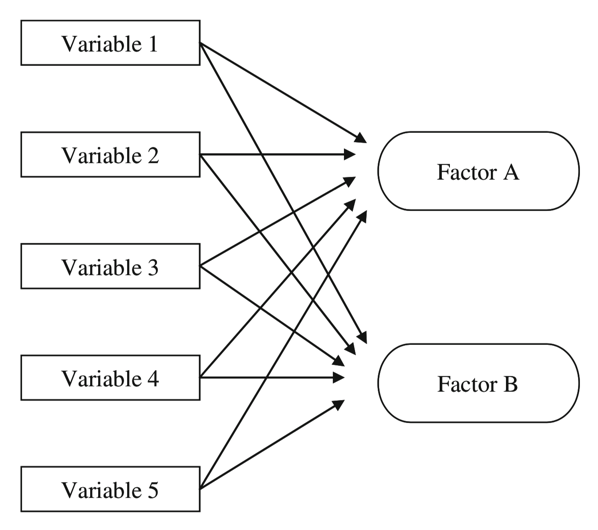## Exploratory factor analysis

Factor Analysis is an exploratory data analysis method used to search influential underlying factors or latent variables from a set of observed variables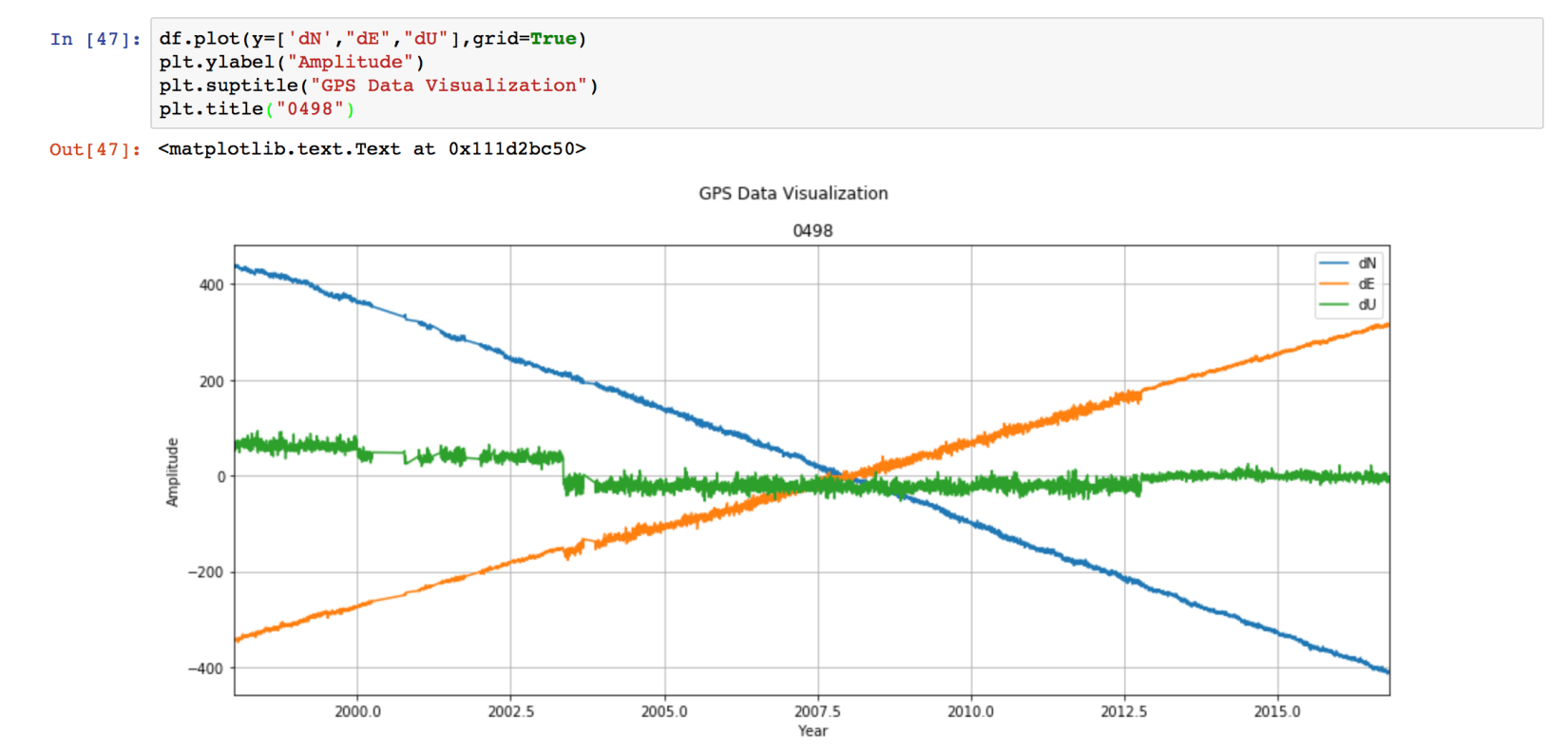## Time series analysis in geophysics

Time-series analysis is essential in most fields of science, including geophysics, economics, etc. Most of the geophysical data comes in a time-series format...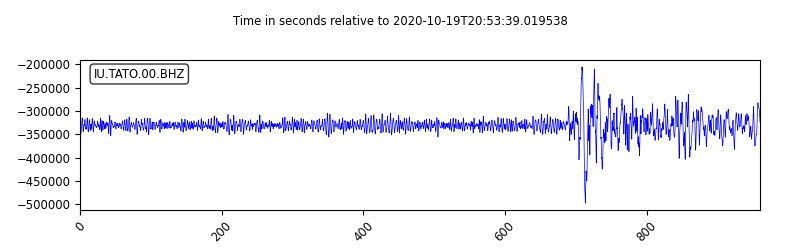Obspy is an open-source Python framework developed for the processing of seismological data. In this post, I will introduce how to use Obspy along with some ...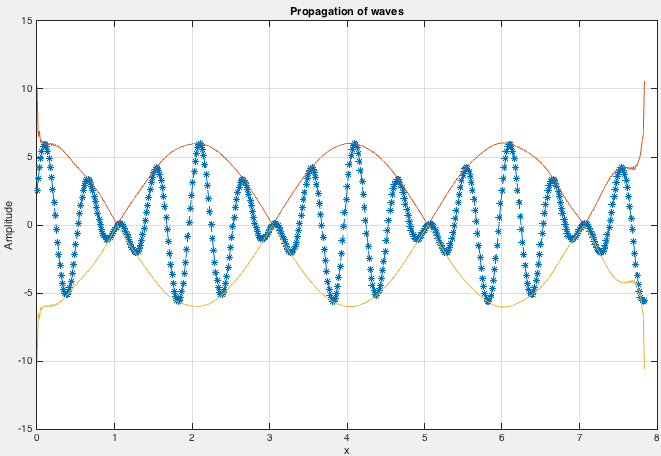## Simple wave modeling and hilbert transform in matlab

We demonstrate how to model a simple wave, obtain its frequencies, apply Hilbert transform, and perform edge detection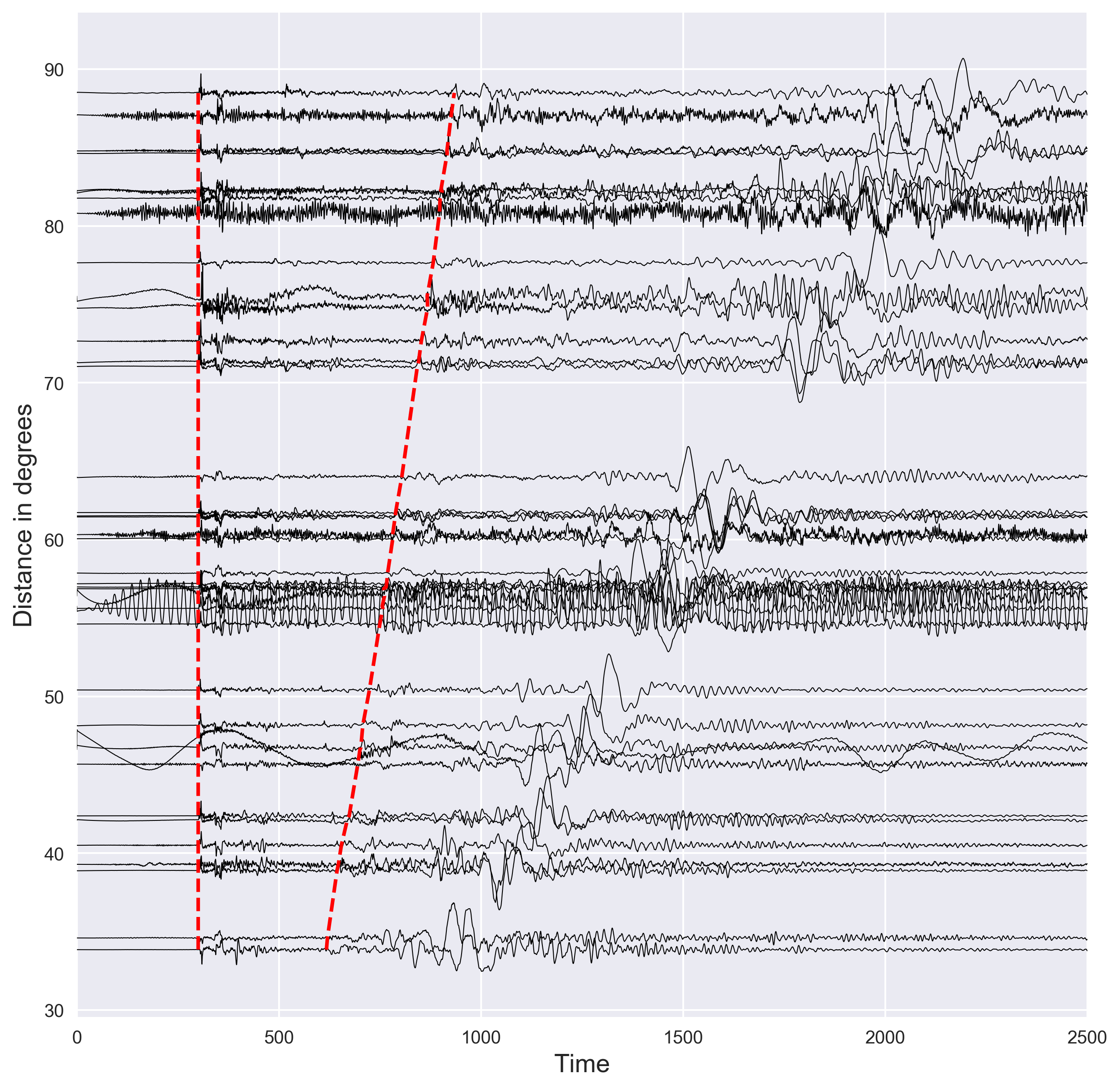## Plotting seismograms with increasing epicentral distance using python

Short demostration of how to plot the distance vs seismic waveforms and mark the P and S arrival times using the IASP91 earth model. Codes are included.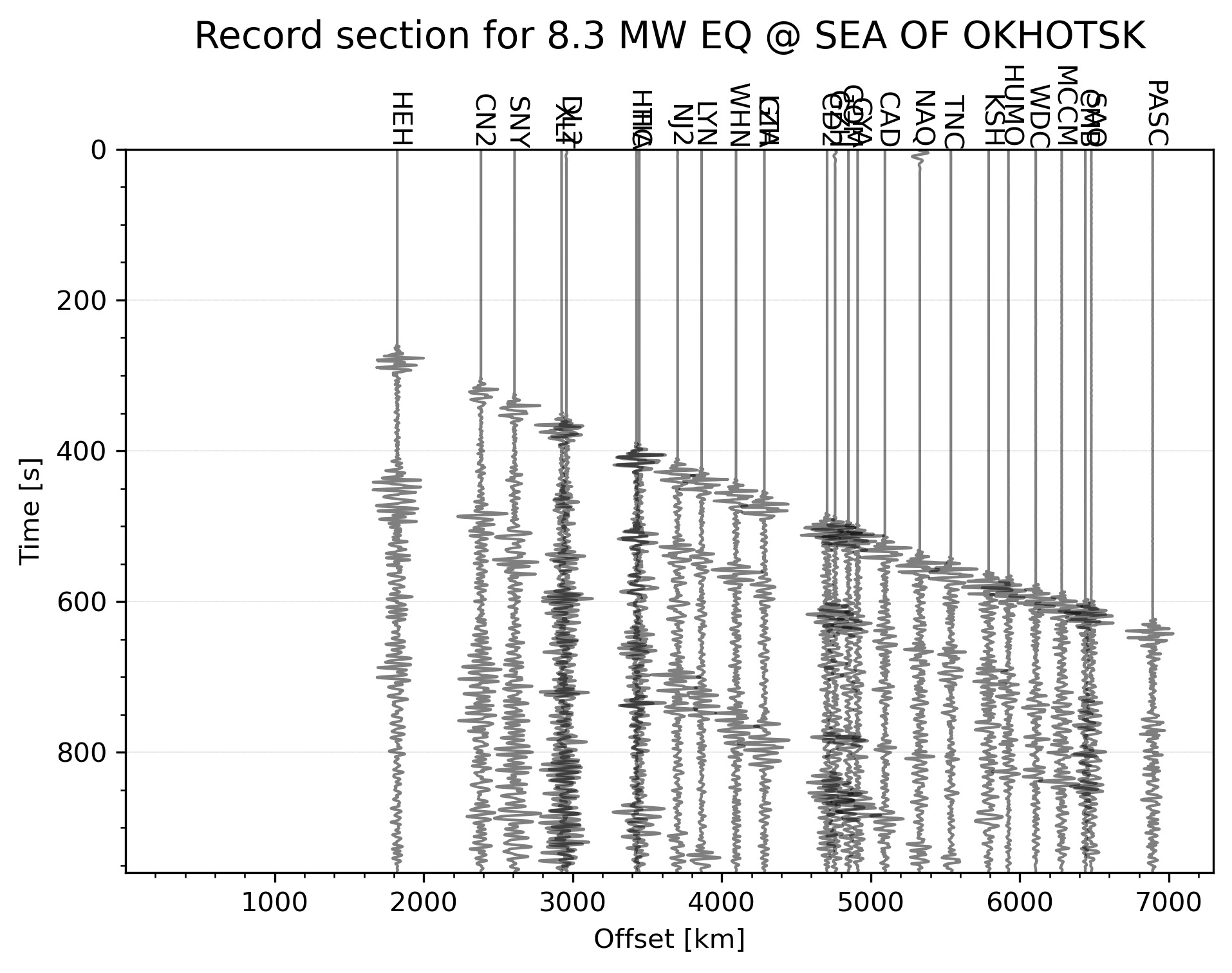## Automatically plotting record section for an earthquake in the given time range [python]

less than 1 minute read      GEOPHYSICS

Python code to automatically plot the record section for the highest magnitude earthquake in the given time range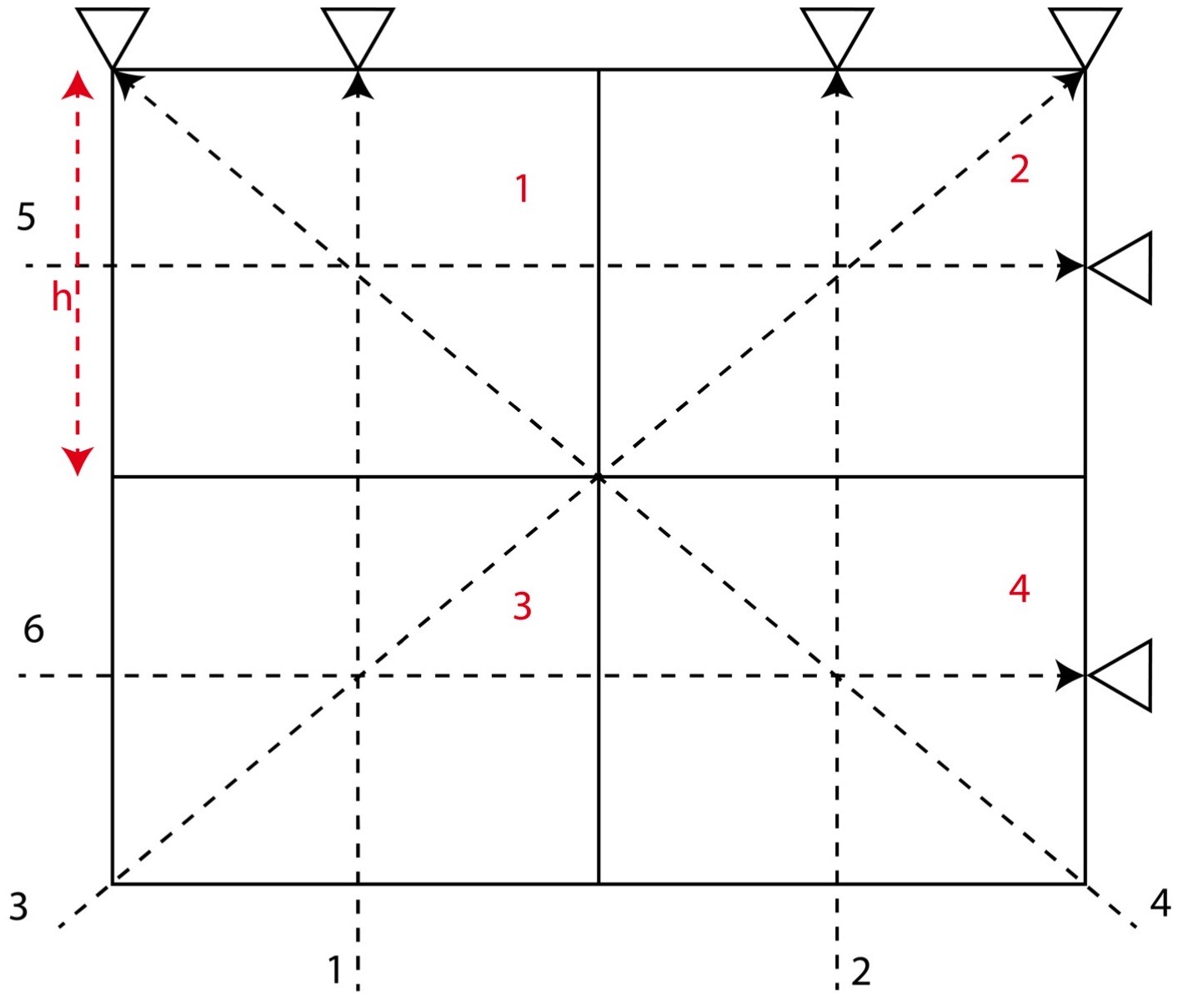## Numerical tests on travel time tomography [matlab]

Introduction to the concepts of tomography with equations and codes. Introduction to the concepts of overdetermined, underdetermined and mix-determined probl...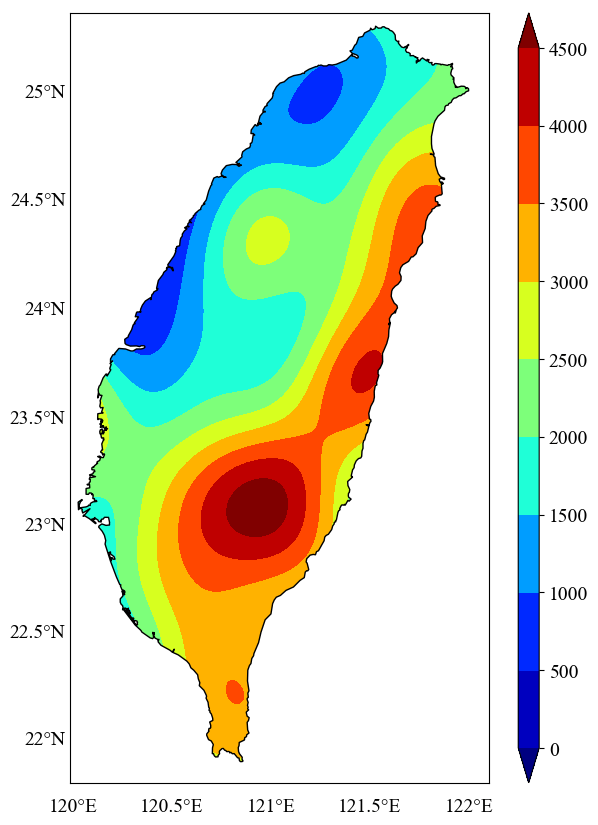## Plotting the geospatial data clipped by coastlines in python

In geosciences, we most frequently have to make geospatial plots, but the available data is unevenly distributed and irregular. We like to show the data, in ...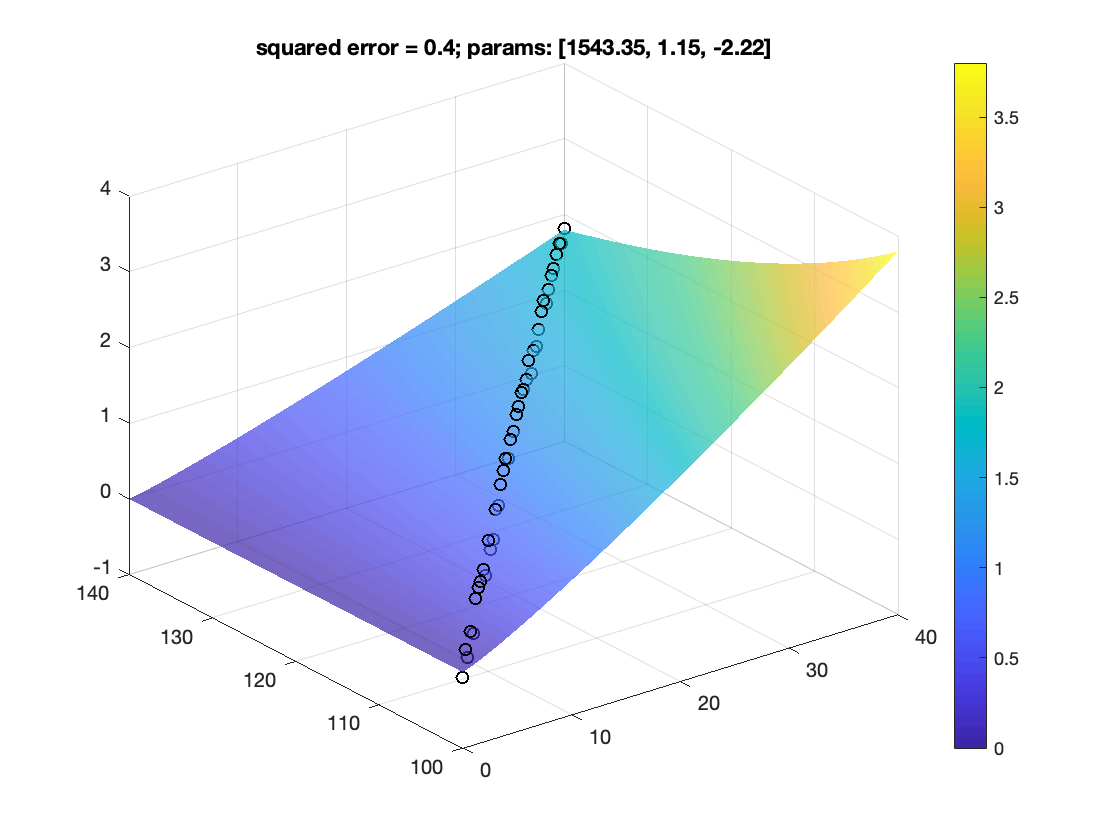## Non-linear curve fitting to a model with multiple observational variables [matlab]

How to fit data to non-linear model

## TECHNIQUES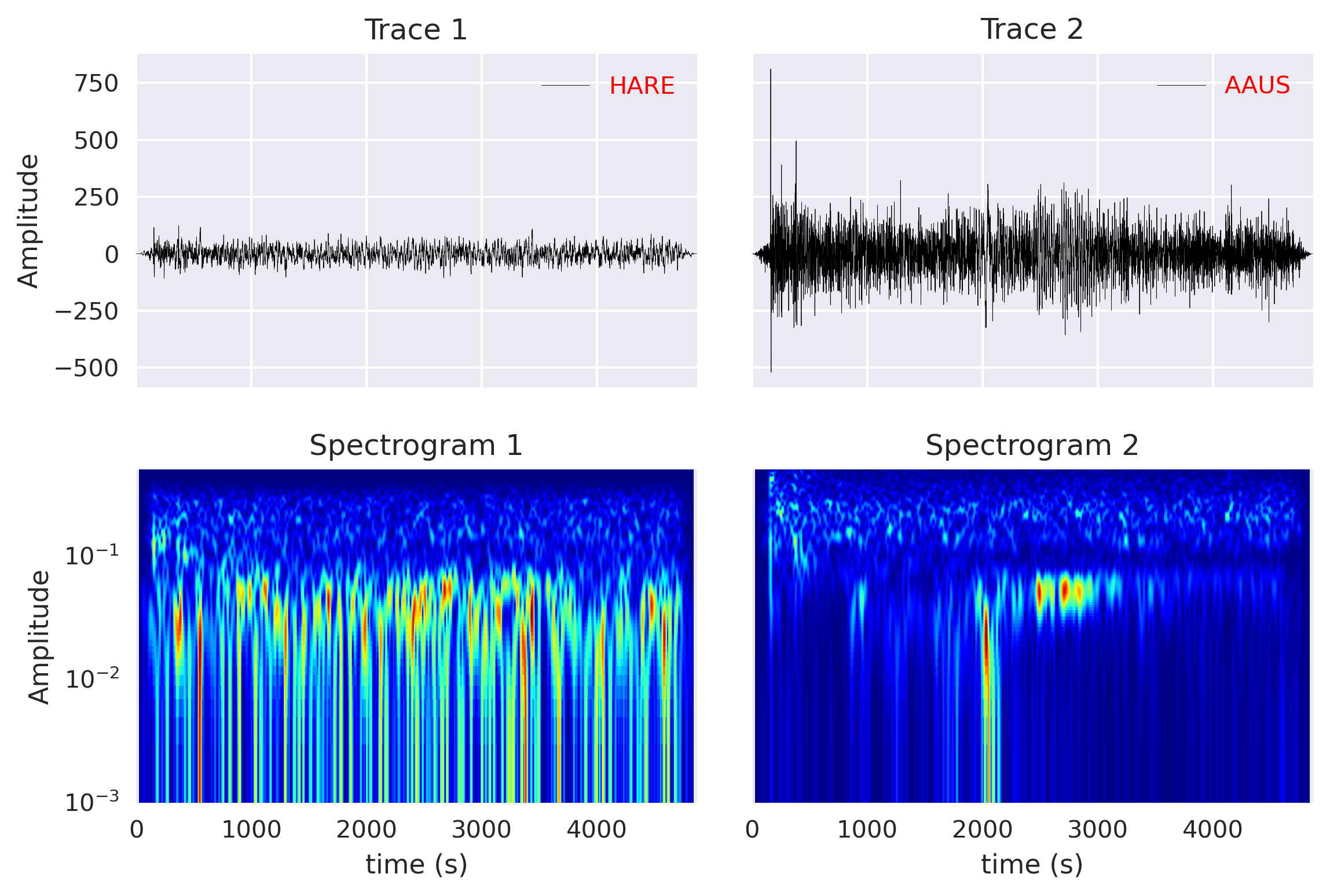## Computing cross-correlation and spectrogram of two seismic traces

Read the seismic traces from the miniseed files and compute the cross-correlation and spectrogram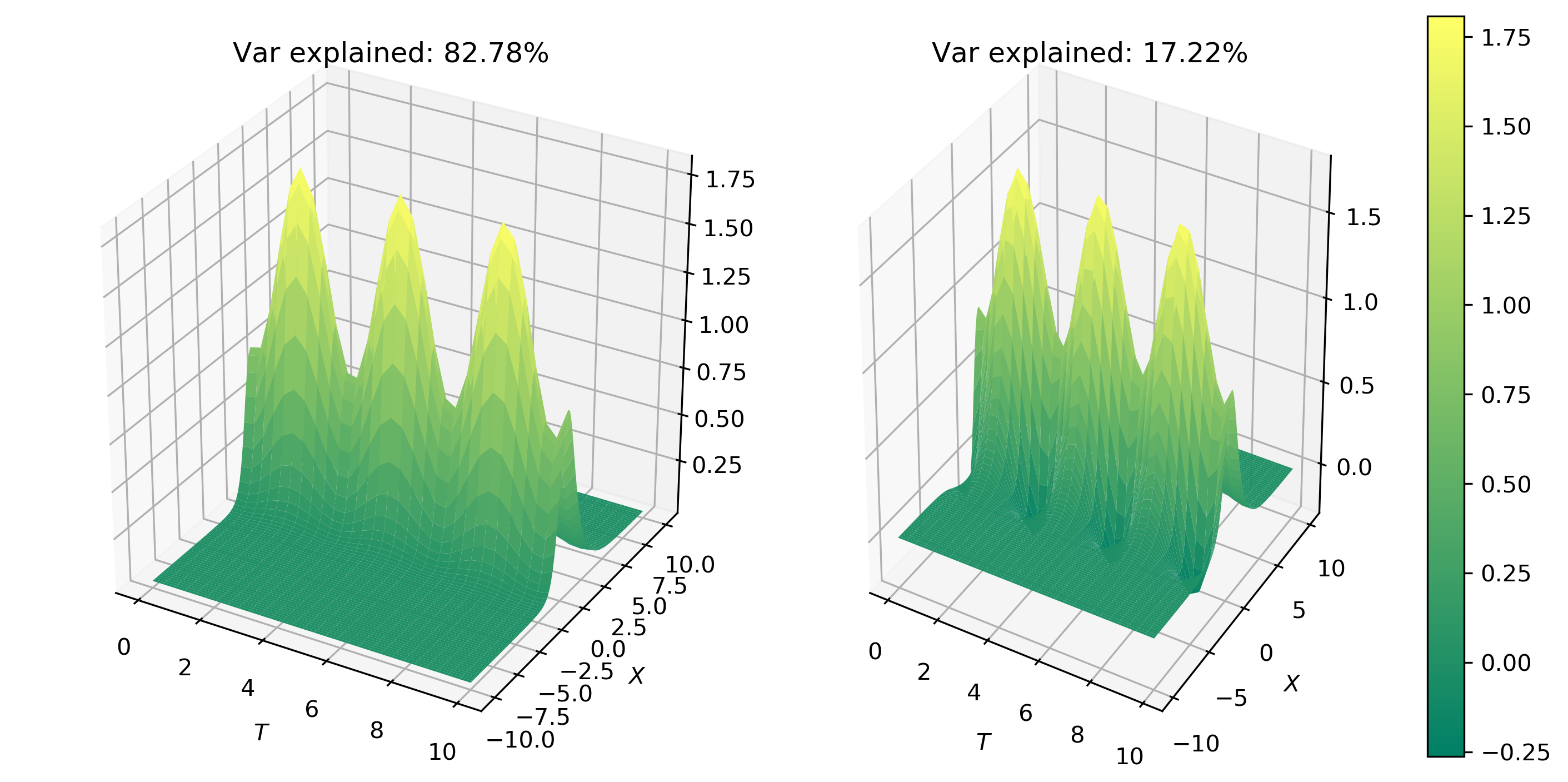## Principal component analysis to decompose signals and reduce dimensionality

We will learn the basics of Fourier analysis and implement it to remove noise from the synthetic and real signals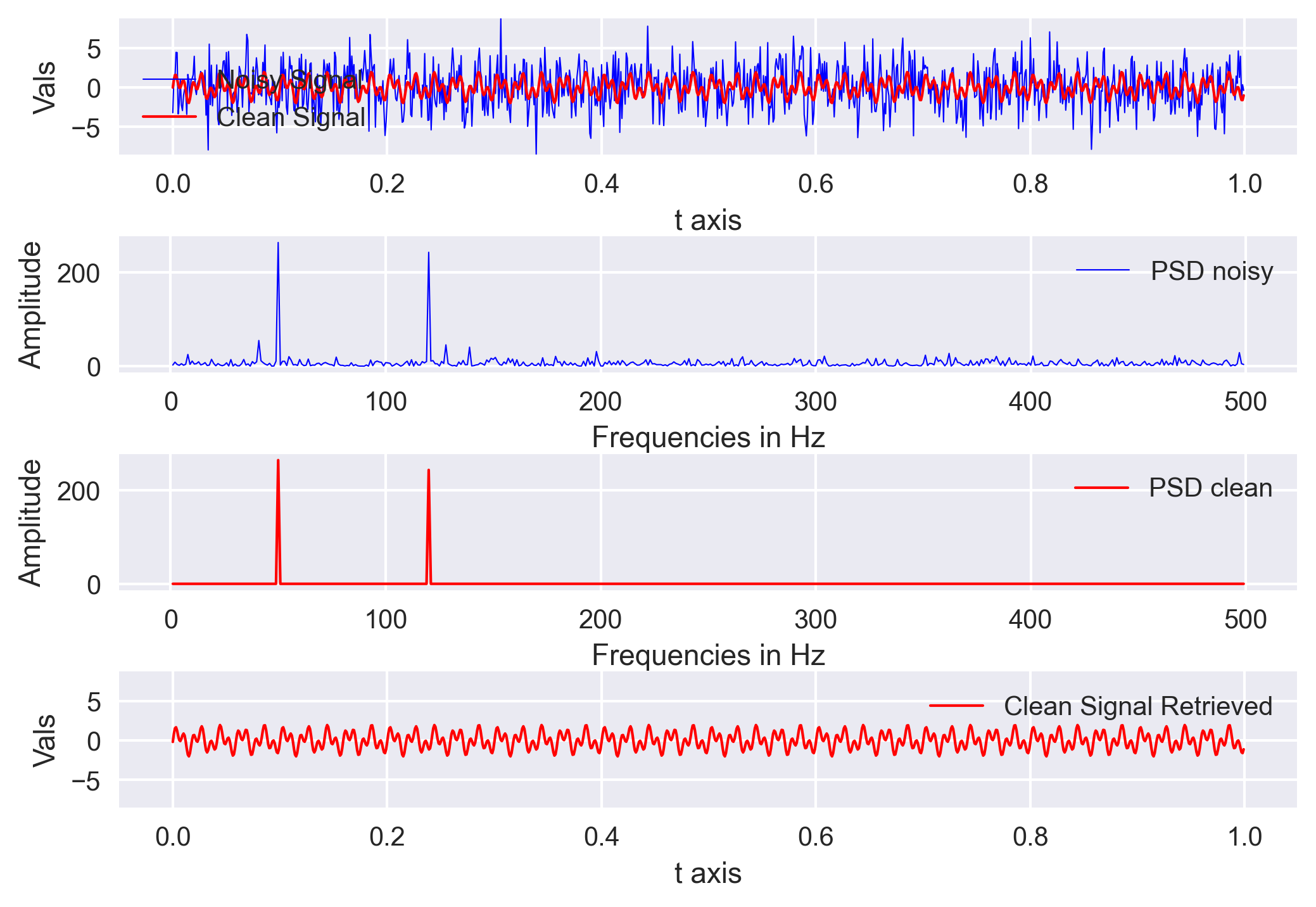## Signal denoising using fourier analysis in python

We will learn the basics of Fourier analysis and implement it to remove noise from the synthetic and real signals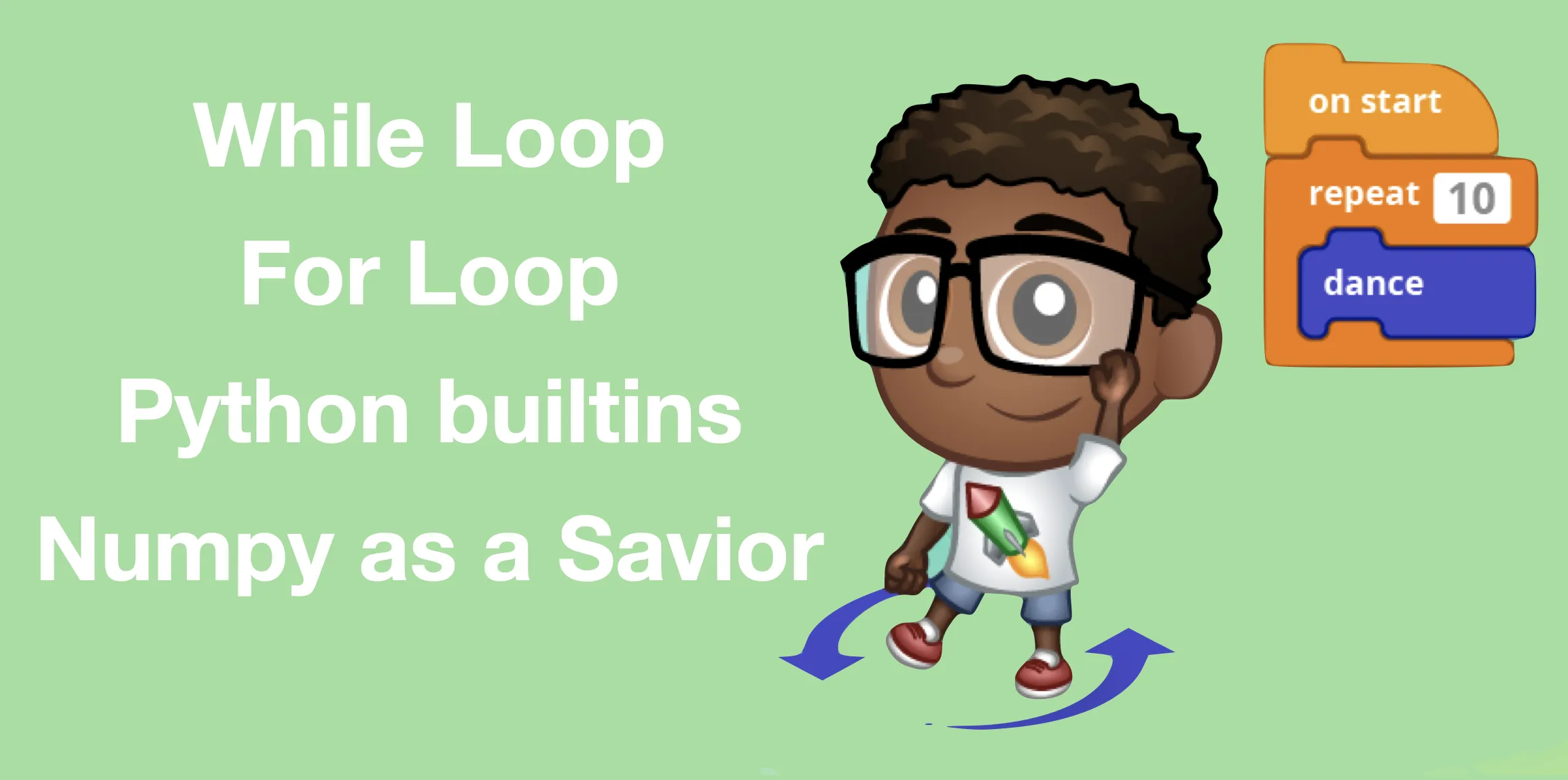## The right way to loop in python

What is the fastest and most efficient way to loop in Python. We found that the numpy is fastest and python builtins are the most memory efficient.## Speed-up your codes by parallel computing in python

Parallel computing is quickly becoming a necessity. Modern computers comes with more than one process and we most often only use single process to do most of...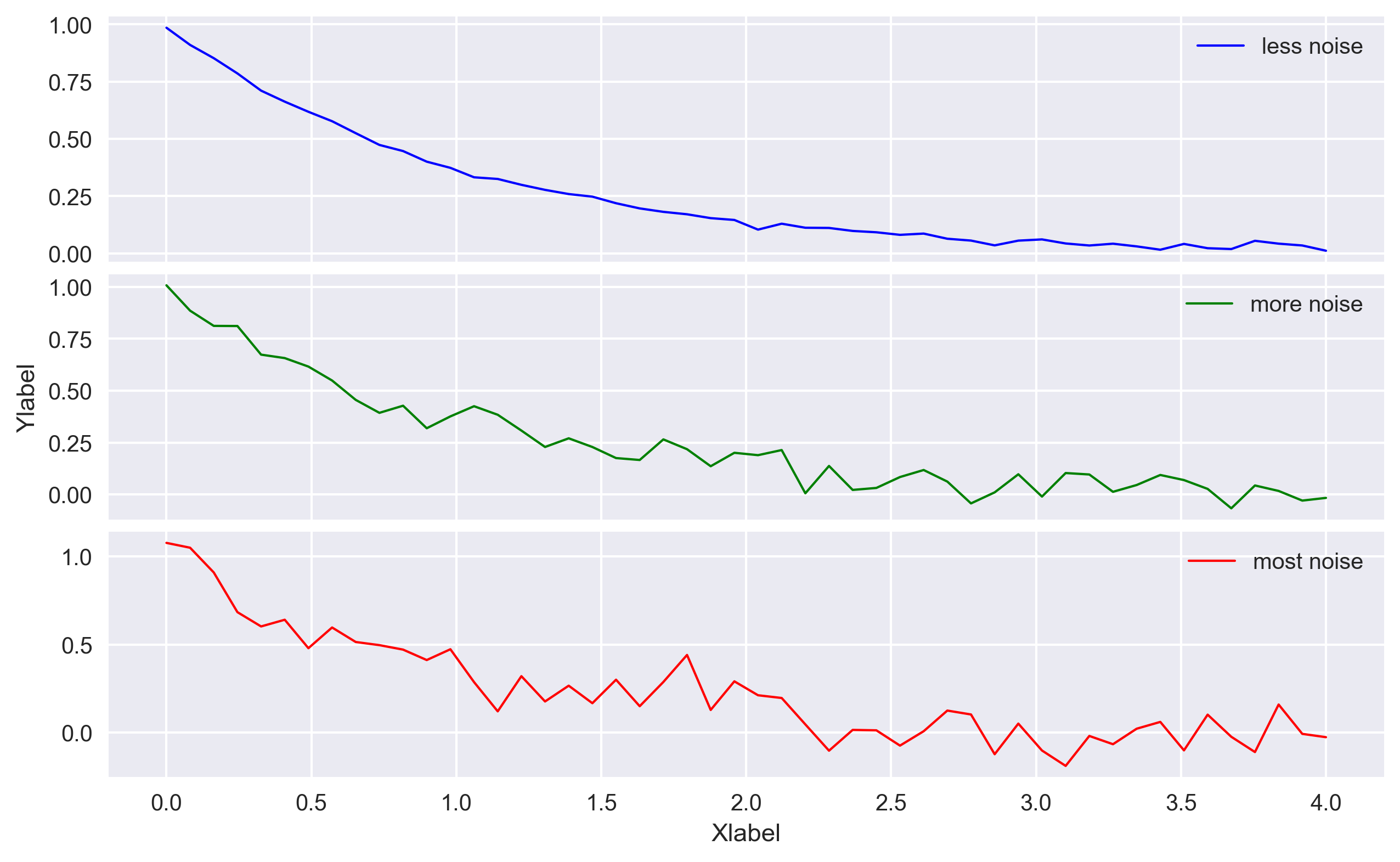## Advanced 2d plots with matplotlib in python

Codes for plotting advanced 2D plots using matplotlib library in Python. Includes simple 2D plot, error bars, bar graphs, histograms, multiple plots, etc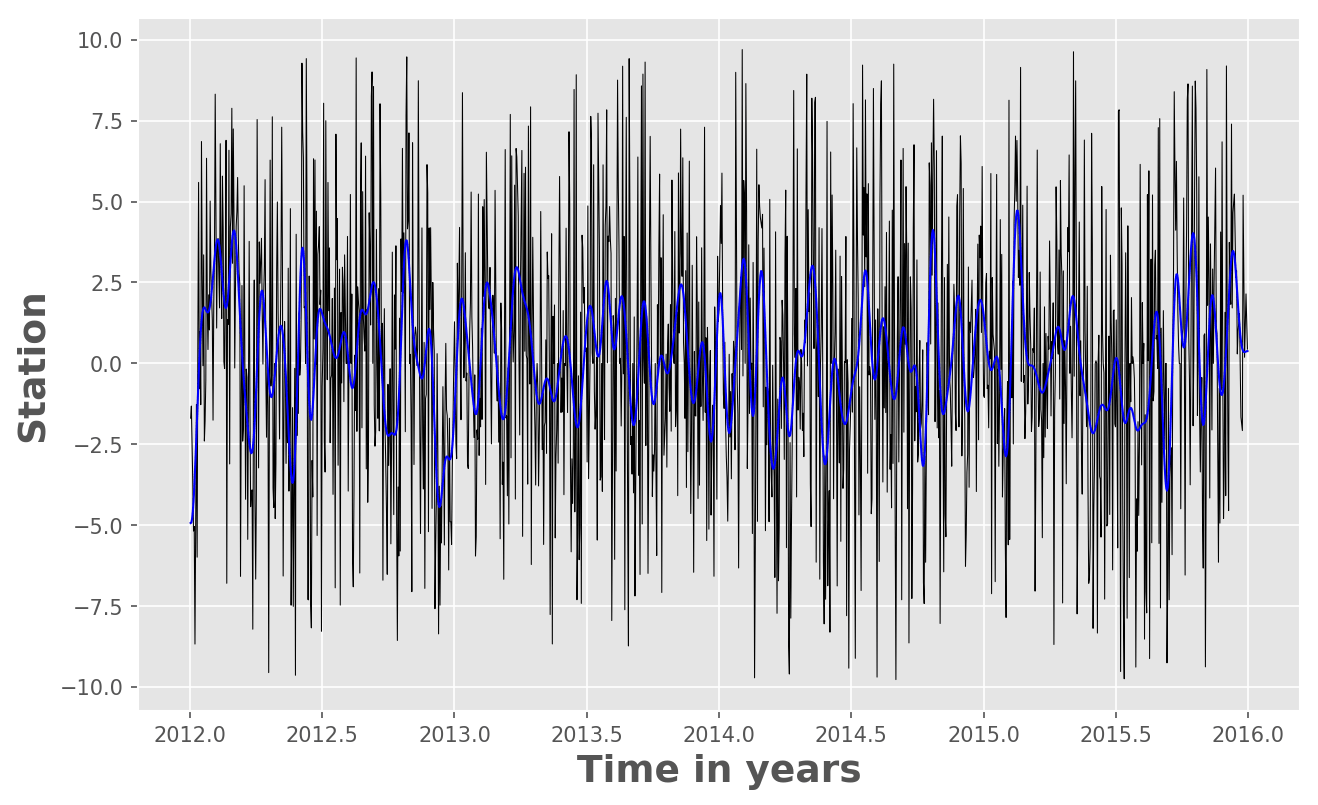## Time series analysis in python: filtering or smoothing data

In this post, we will see how we can use Python to low pass filter the 10 year long daily fluctuations of GPS time series. We need to use the “Scipy” package...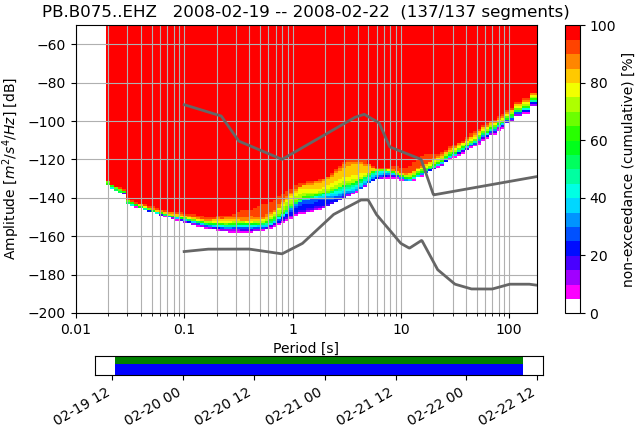## Visualizing power spectral density using obspy [python]

Short demonstration of the ppsd class defined in Obspy using 3 days of data for station PB-B075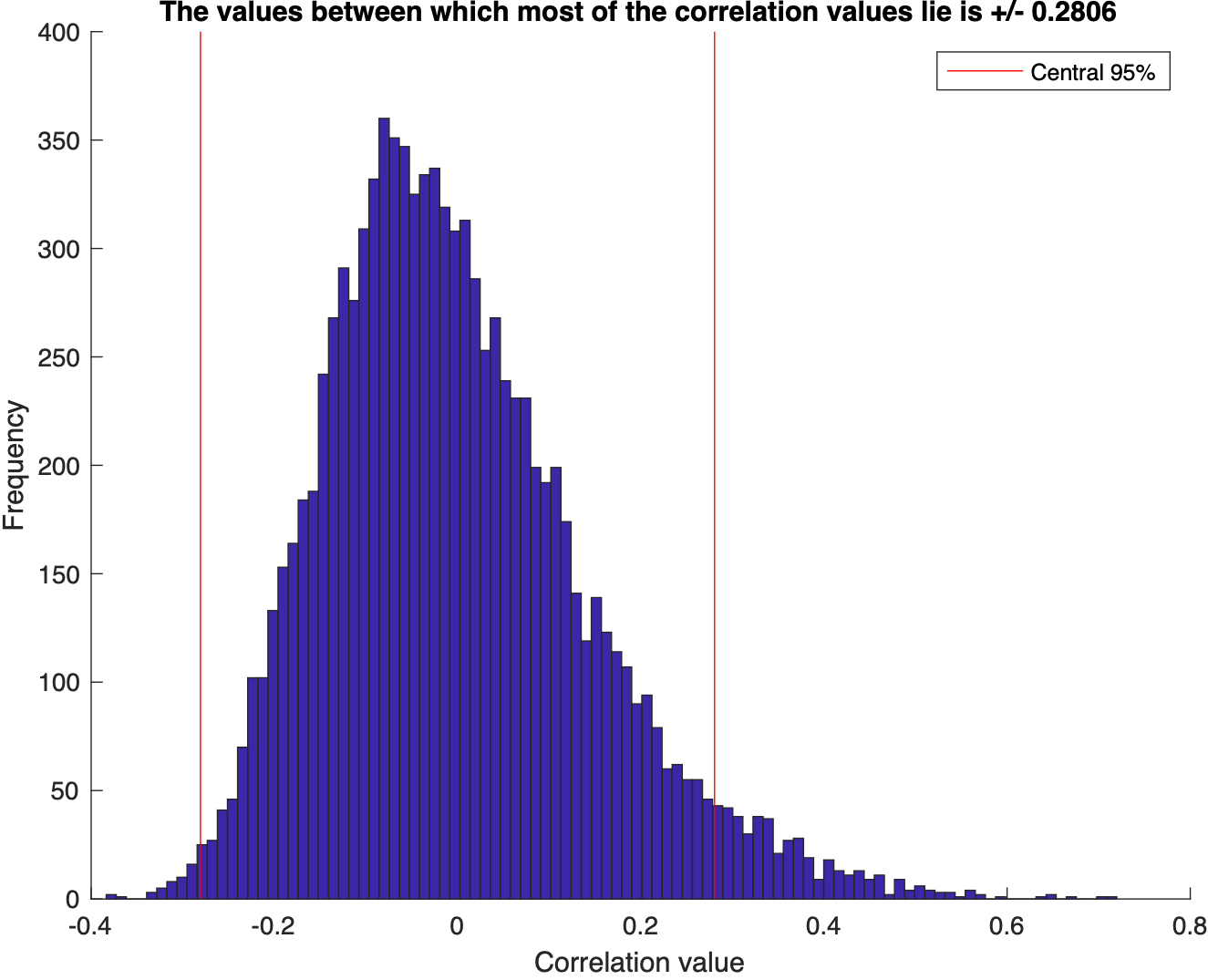## Monte carlo simulation to test for the correlation between two dataset [matlab]

We test for the correlation coefficients or the covariance between two sets of random numbers selected from normal distribution using the Monte Carlo simulat...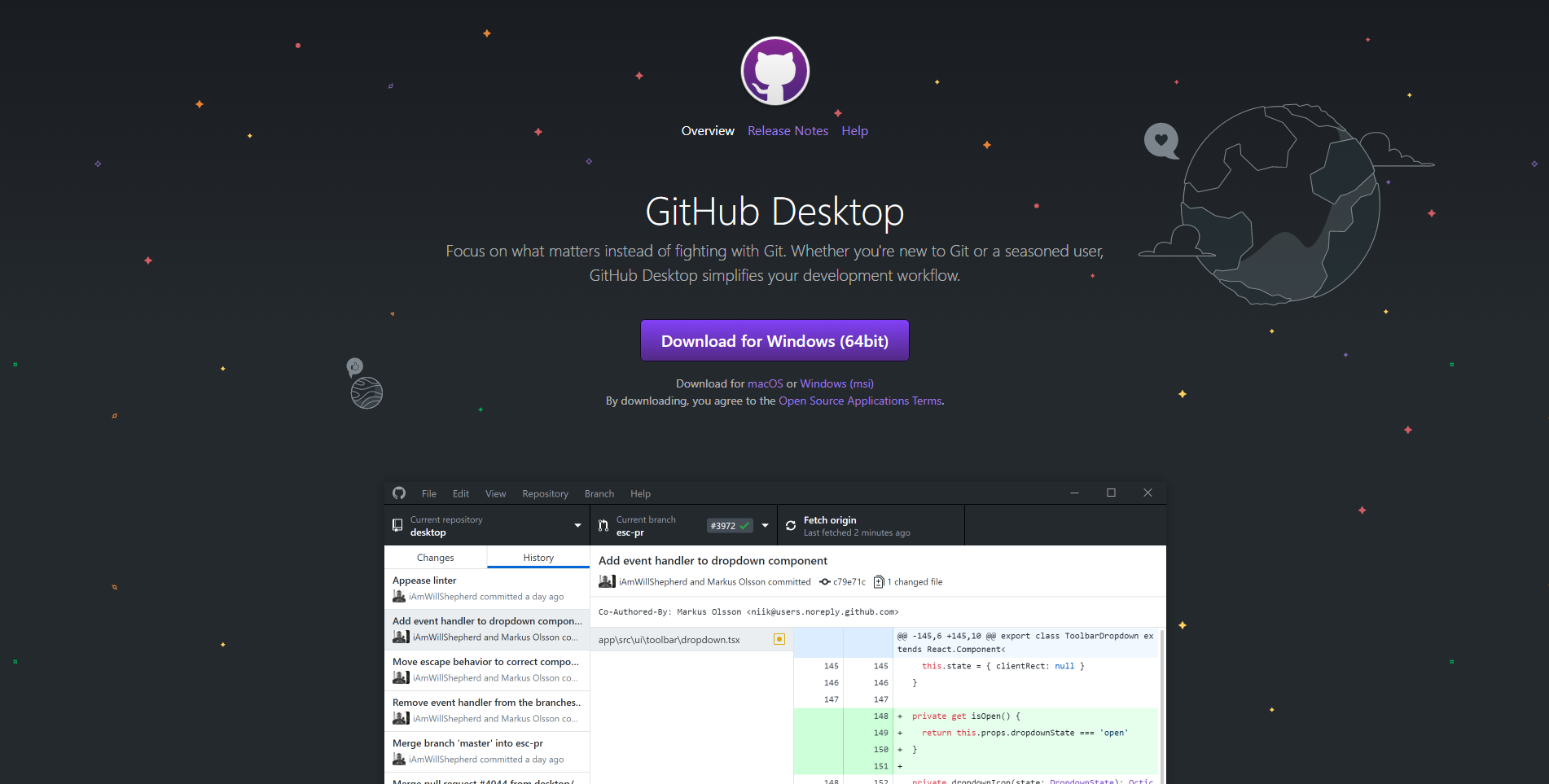## Using github for team collaboration

Tutorial on how to use Git and GitHub for team collaboration on a project. Content includes installing, setting up, creating a repository, making commits, un...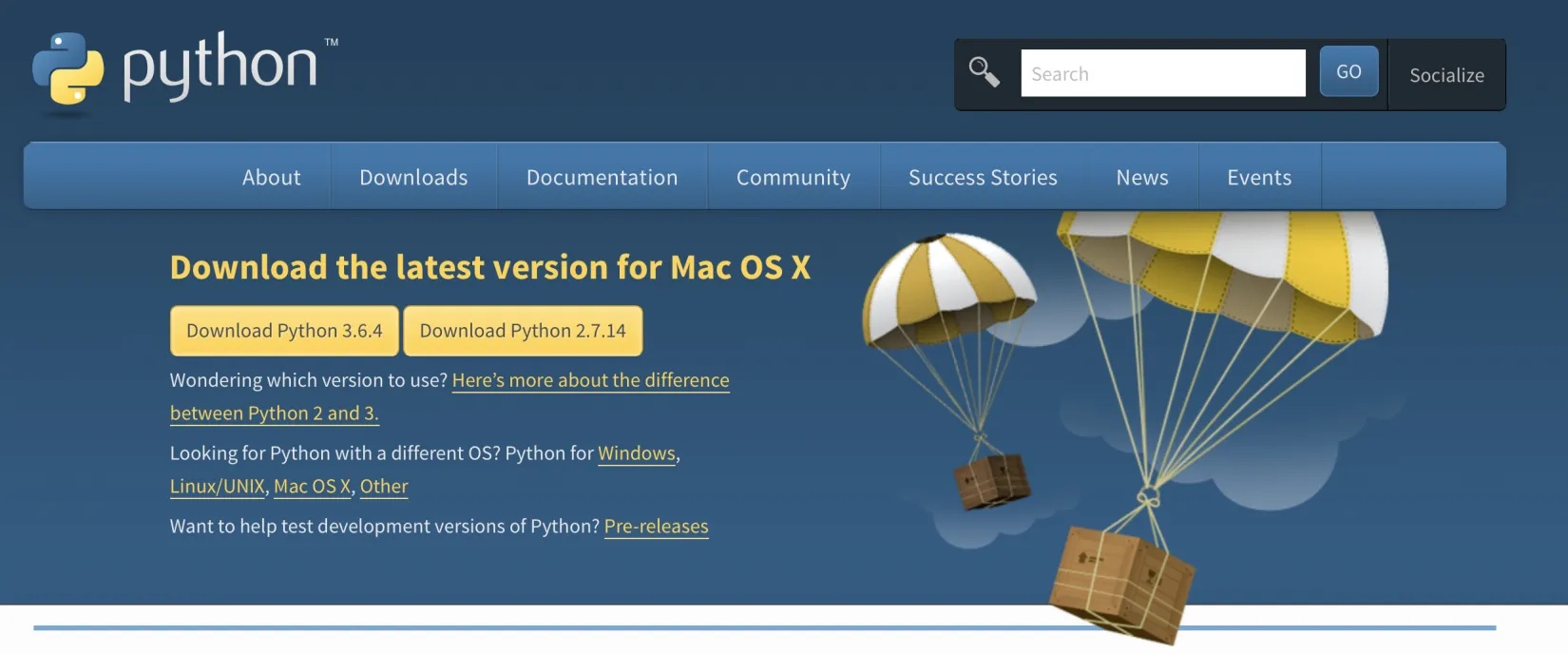## Introduction to python for beginners

In this tutorial post, I give a quick demo of how to install Python (using anaconda) and then getting started with writing simple scripts.

## STATISTICS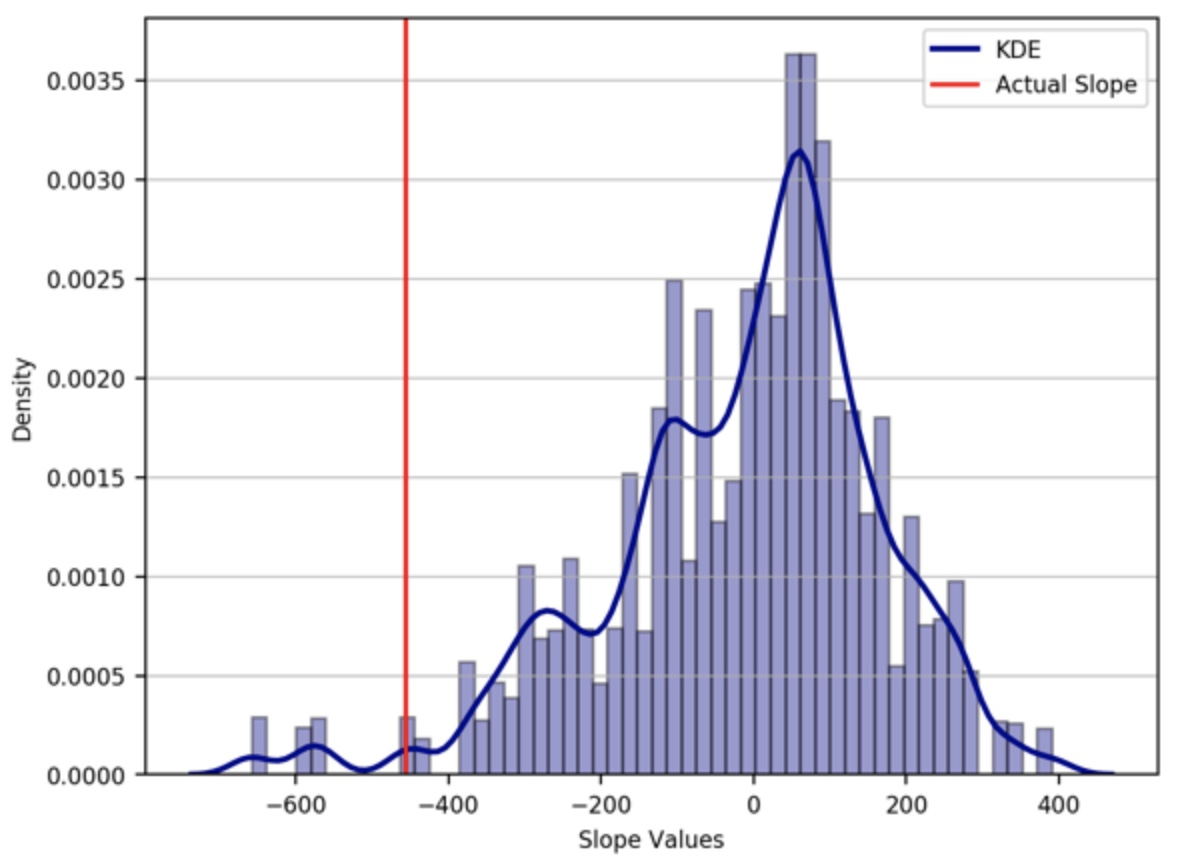## Hypothesis test for the significance of linear trend using the monte carlo simulations

We pose a null hypothesis and enquire that given that the null hypothesis is true, how likely is the observed pattern of results? This likelihood is known as...## Easy statistical analysis using the tools of matlab

Visualize the statistics of the data using MATLAB: mean, median, std, interquartile range, skewness, kurtosis, t-statistic, degrees of freedom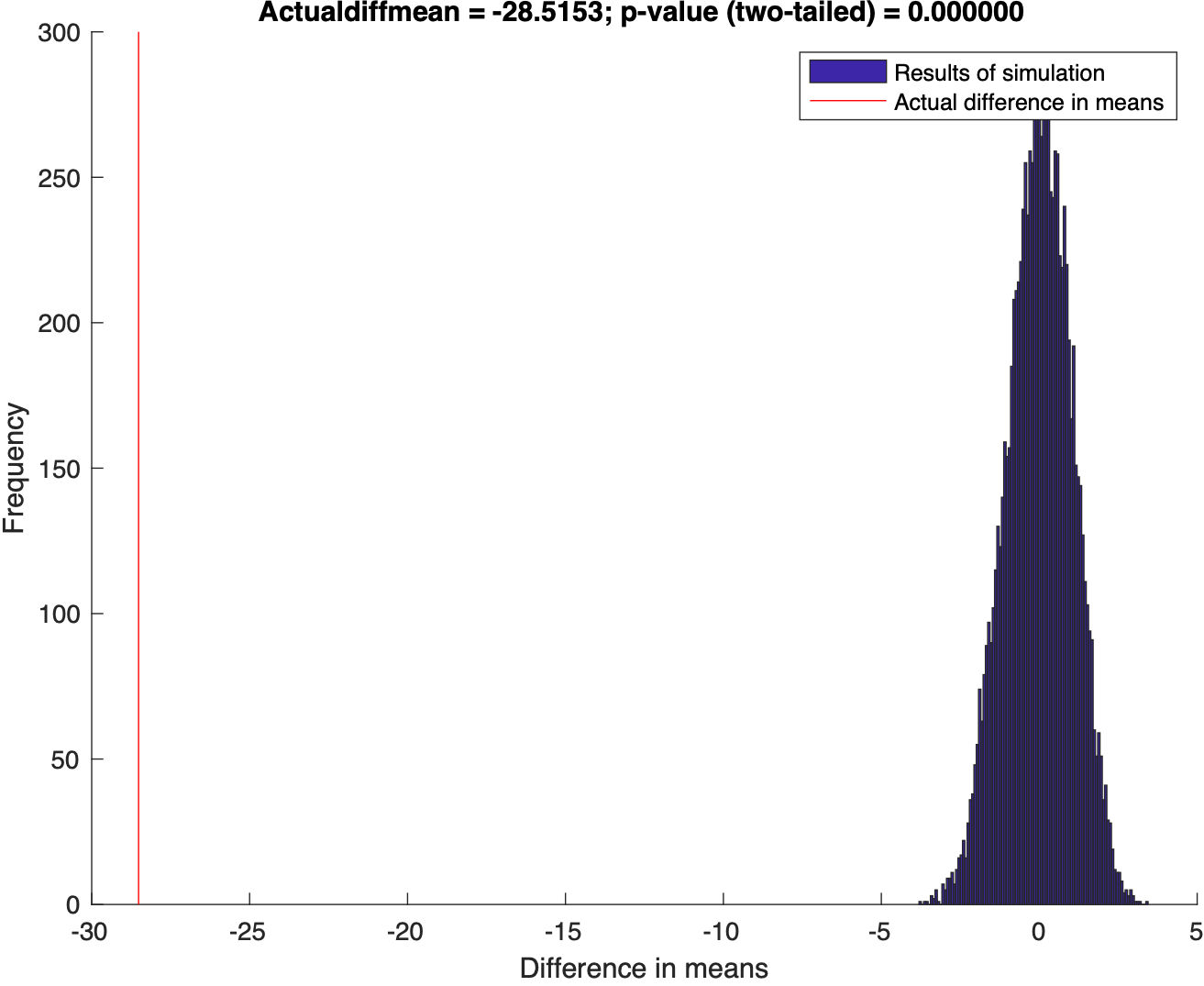## Hypothesis test [matlab]

Using Randomization to test the disprove the null hypothesis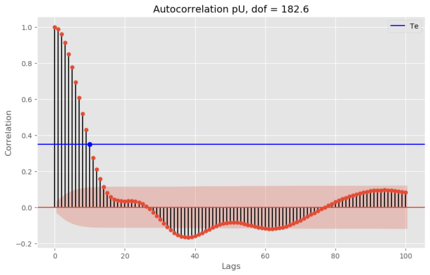## Estimation of the degrees of freedom of time series [python]

Two time series with predominant linear trends (very low DOF) can have a very high correlation coefficient, which can hardly be construed as an evidence for ...

## LIFEHACKS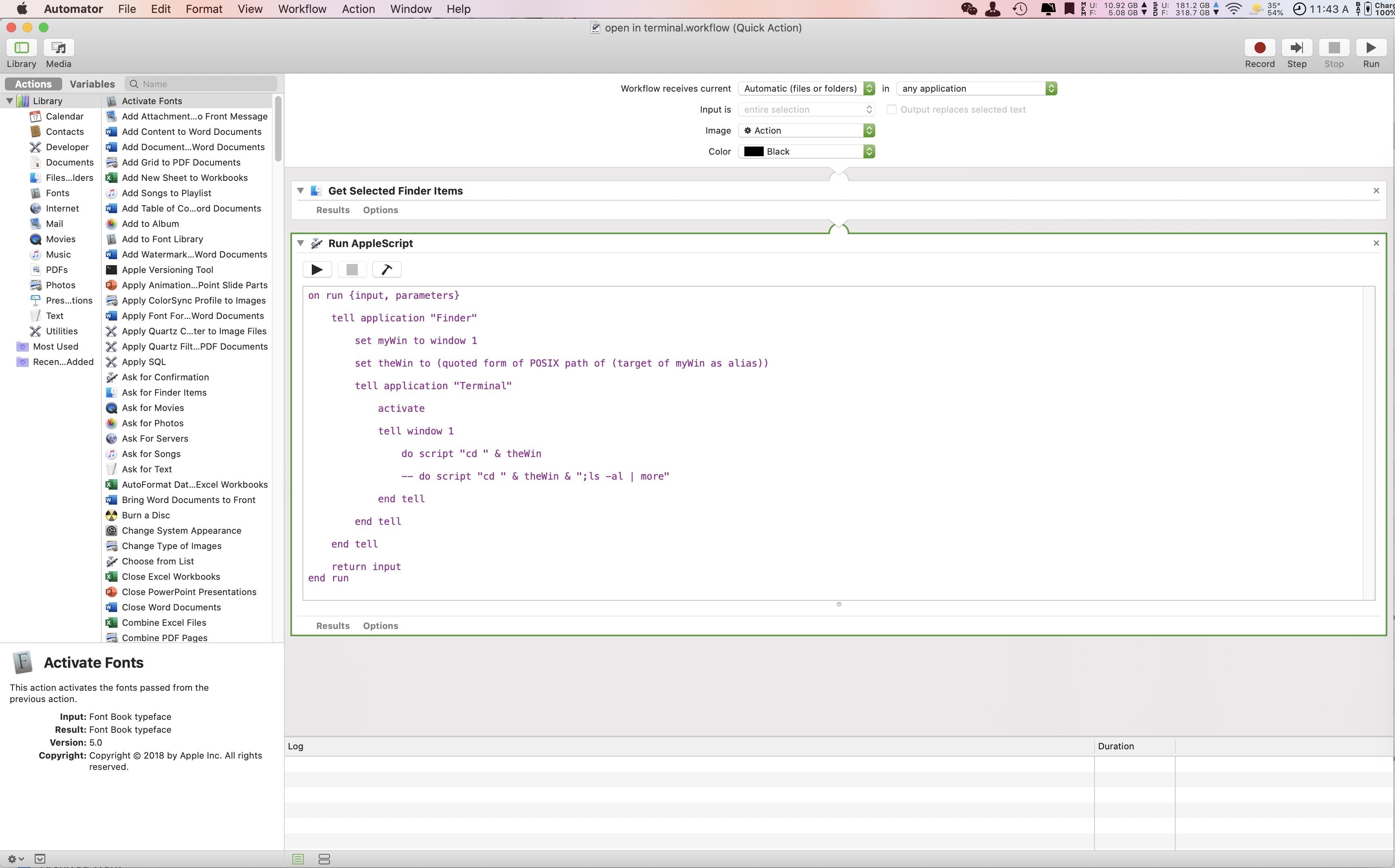## Some handy mac tweaks [macos]

Some handy tweaks for mac like relocating default screenshot location, renaming batch files etc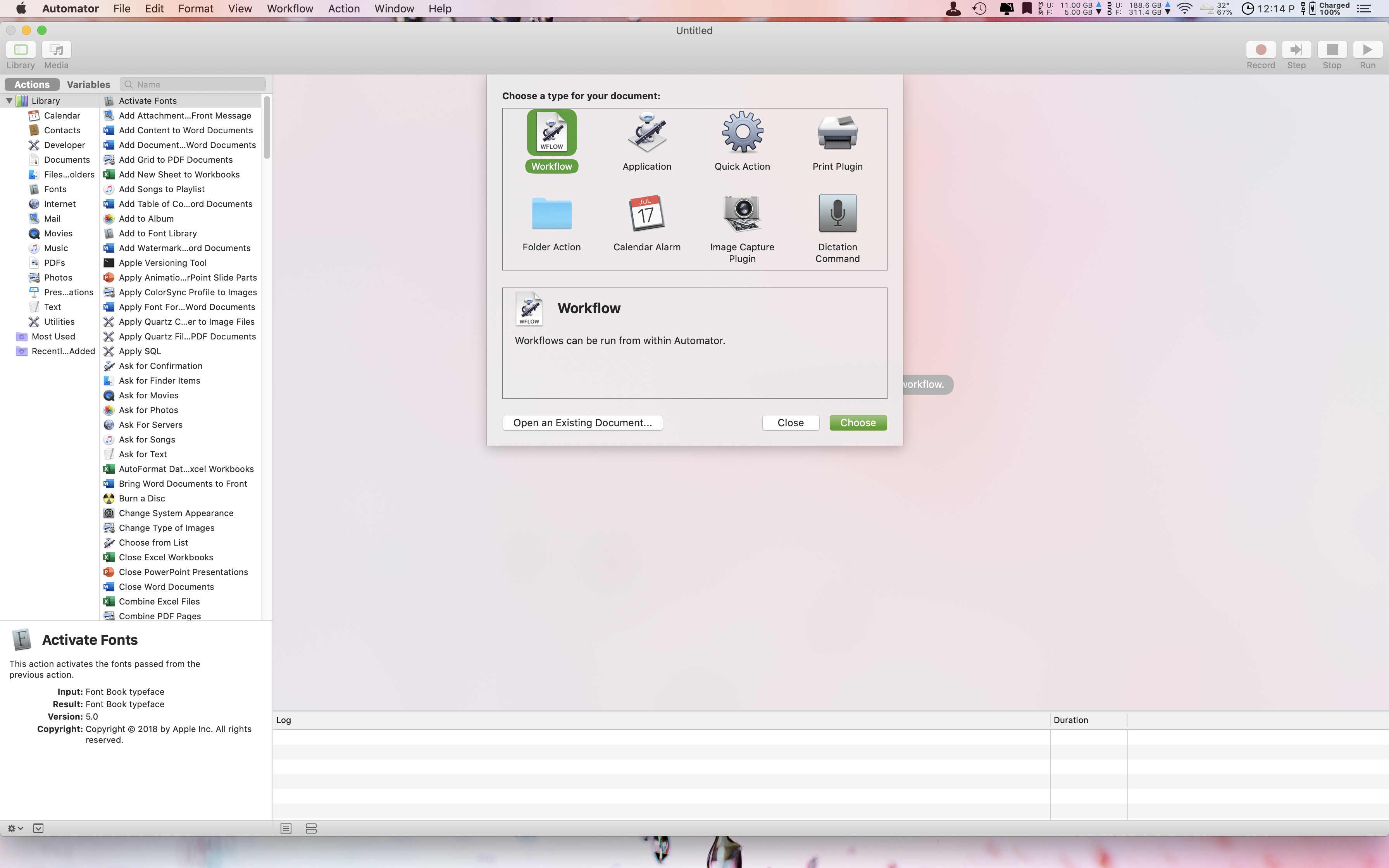## Introduction to automating mac [macos]

Mac can be easily automated by the help of several tools such as automator, quick actions, applescripts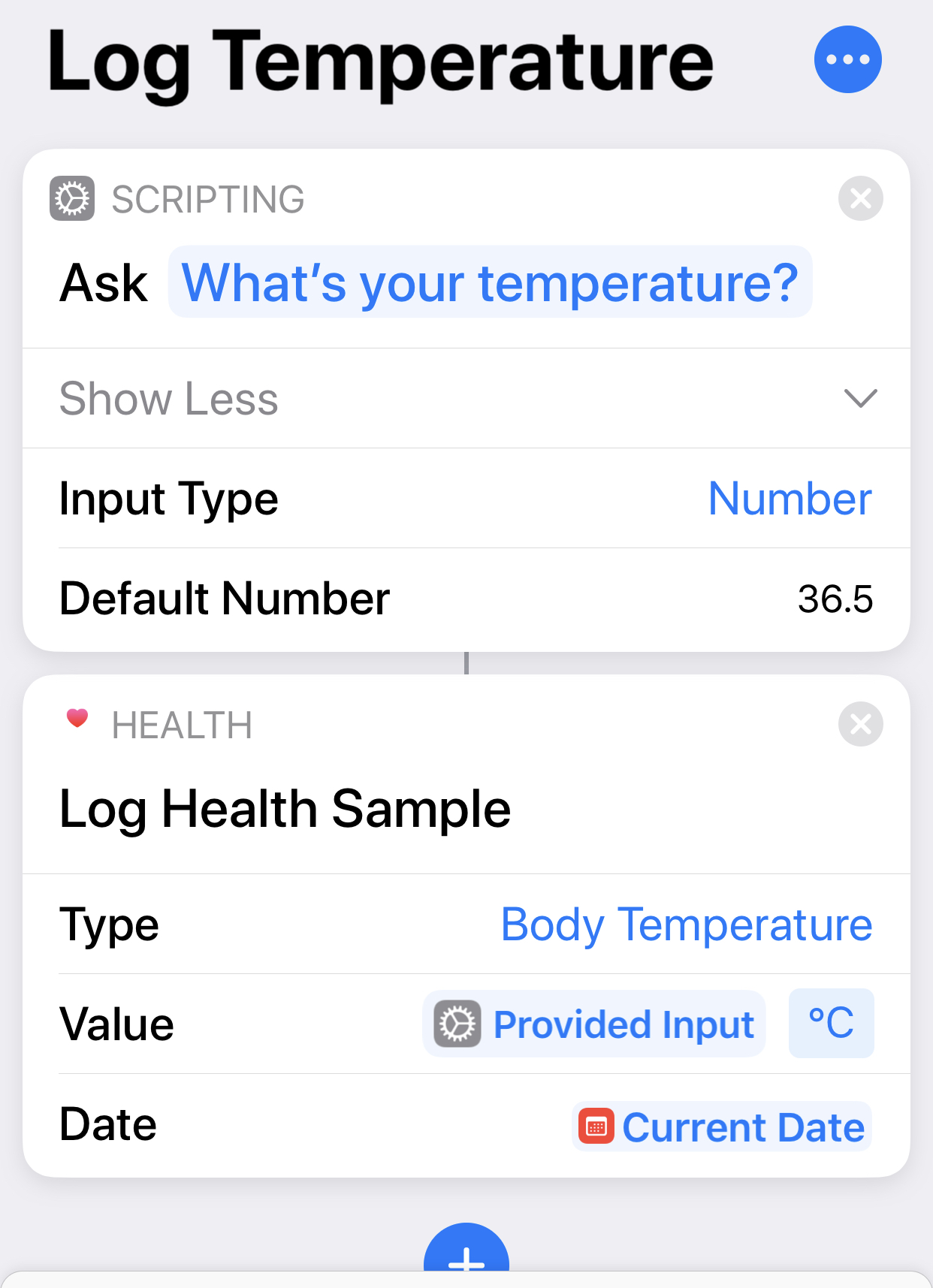## Iphone shortcut to quickly log body temperature [ios]

Shortcut code for quickly logging temperature in Apple health app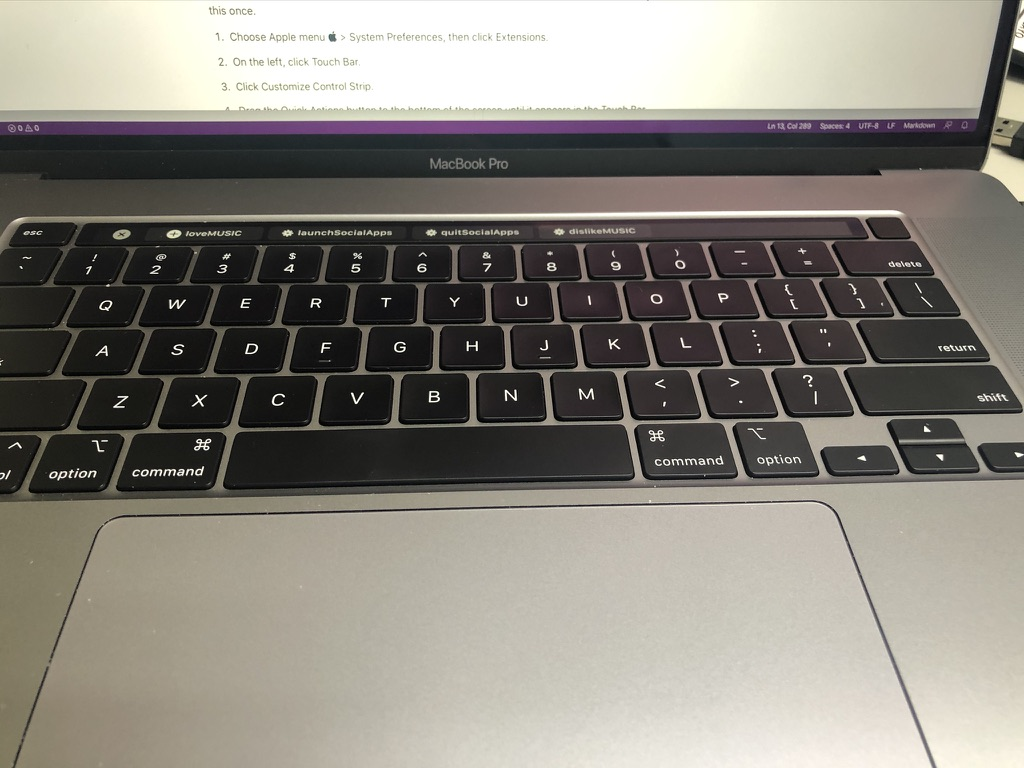## Mac quick action to rate the songs in apple music app [macos]

Quick action for mac to easily love, dislike, rate songs in apple music app

## MACHINELEARNING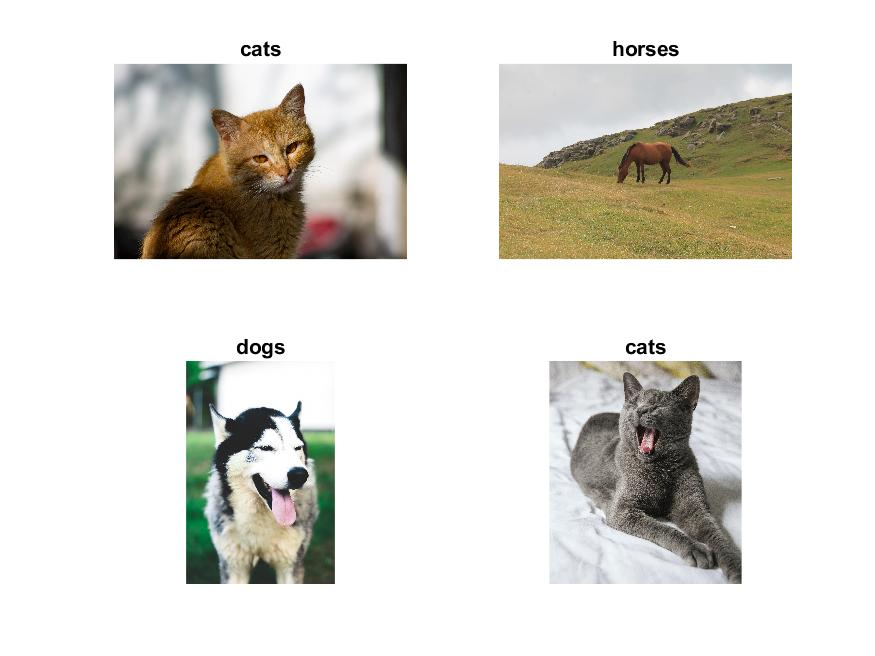## Transfer learning applied on the unsplash data using alexnet pretrained network

Transfer learning using the pre-trained deep learning networks from MATLAB can be easily implemented to achieve fast and impressive results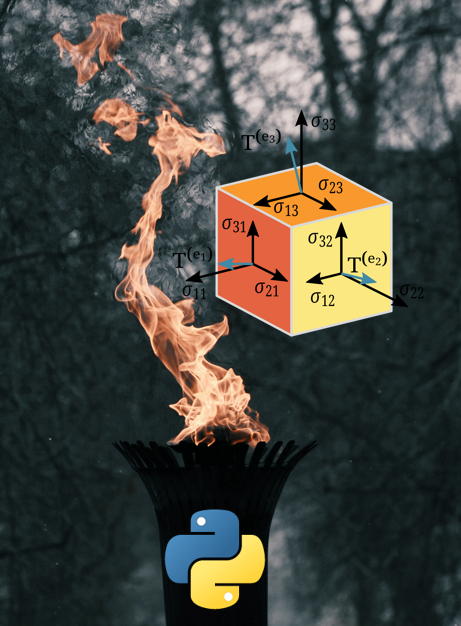## Getting familiar with pytorch data structures

In this introduction to the concepts of Pytorch data structures, we will learn about how to create and reshape tensors using Pytorch and compare it with the ...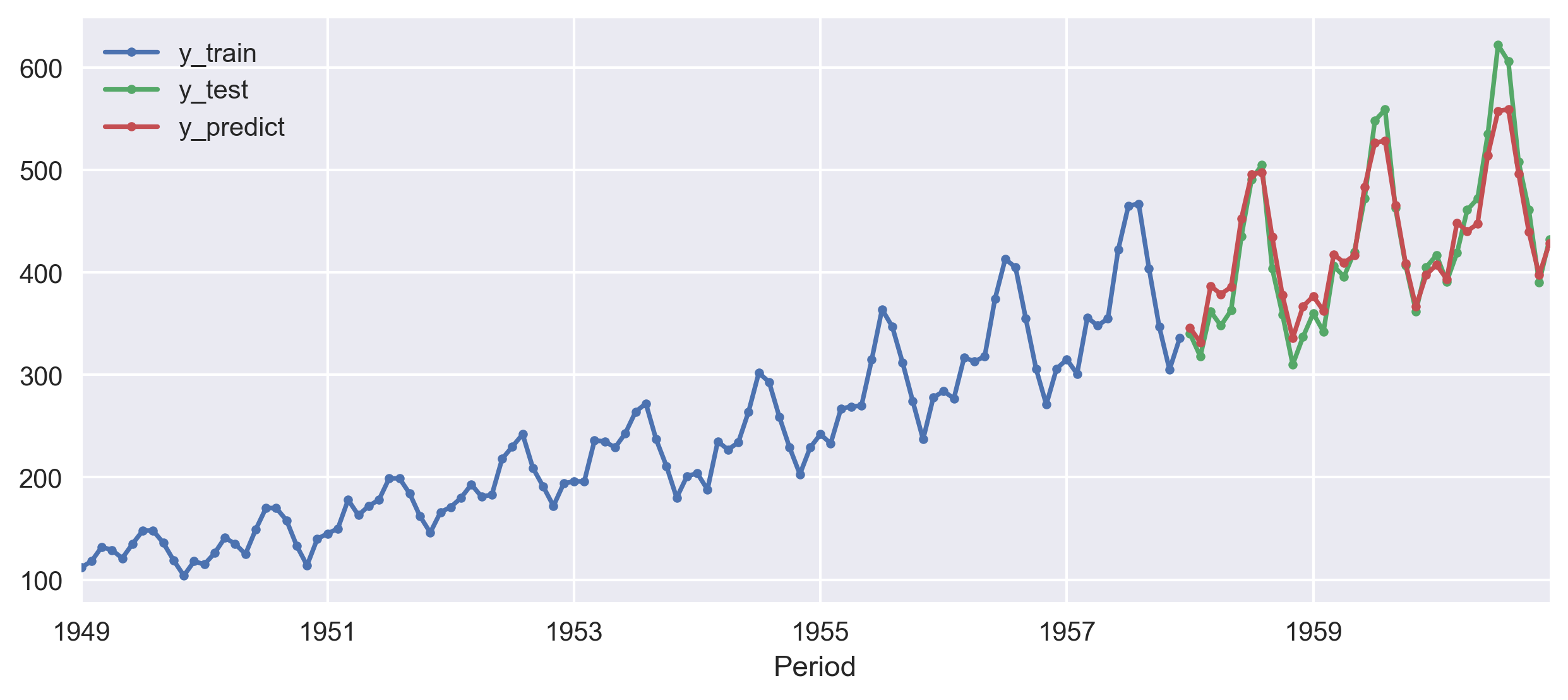## A quick look into the sktime for time-series forecasting

I used the sktime library to forecast the airline data using NaiveForecaster, KNeighborsRegressor, Statistical forecasters, and auto ARIMA model.

## WEBAPPS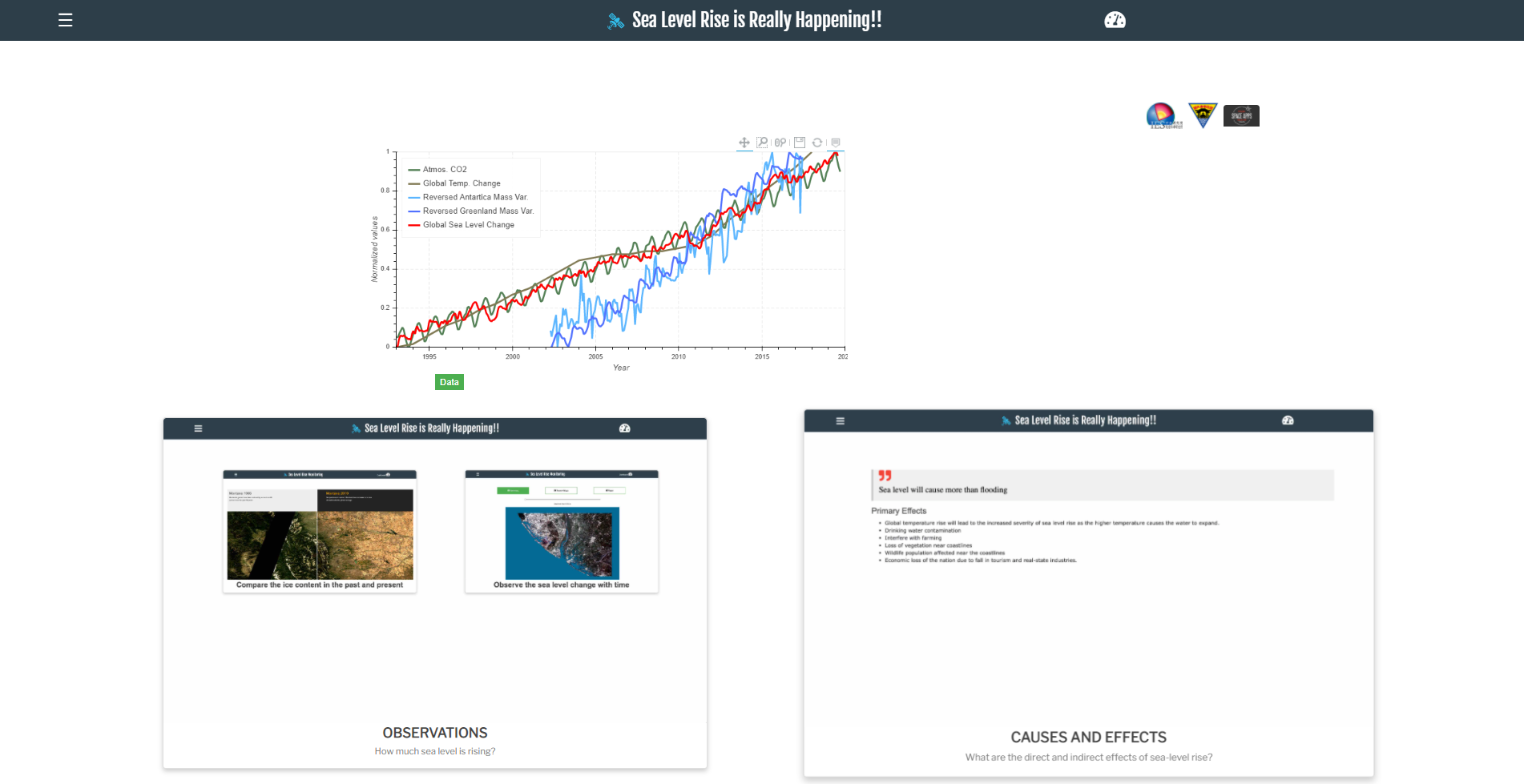## Build a flask web application: sea level rise monitoring

This post gives a quick introduction on how to build a web application using Flask and deploy on Heroku server. Then, I share my codes for building advanced ...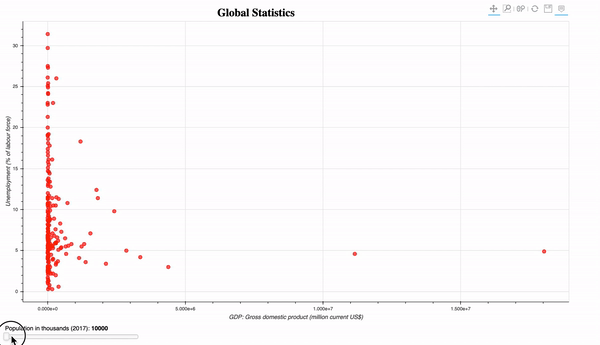## Interactive data visualization in python using bokeh

A basic to advanced guide to making interactive plots in Bokeh.

## DESKTOPAPPS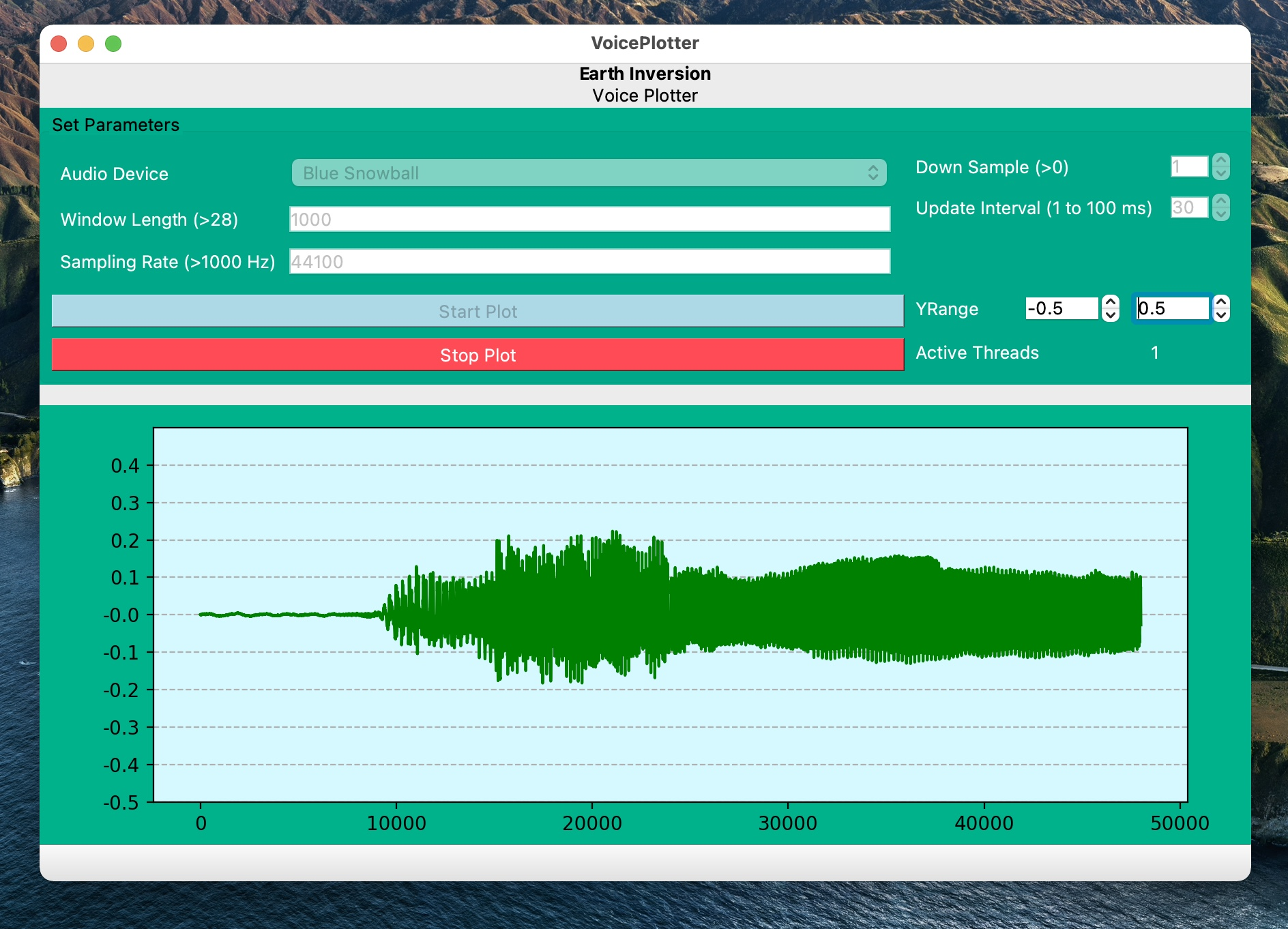## Pyqt5 application for visualizing ambient sound in real-time